# RD Sharma Solutions for Class 6 Maths Chapter 4: Operations on Whole Numbers

Whole Numbers are very important in our daily lives to obtain the exact count of things which are used by us. It is therefore important for students to gain knowledge about concepts which are covered in this Chapter. The subject experts create solutions having explanations in a simple language based on the understanding capacity of students.

The primary objective of preparing solutions is to provide students with chapter wise solutions which improves knowledge about concepts. RD Sharma Solutions contain problems solved in a comprehensive manner based on the CBSE guidelines and exam pattern. RD Sharma Solutions for Class 6 Maths Chapter 4 Operations on Whole Numbers are provided here.

## RD Sharma Solutions for Class 6 Maths Chapter 4: Operations on Whole Numbers Download PDF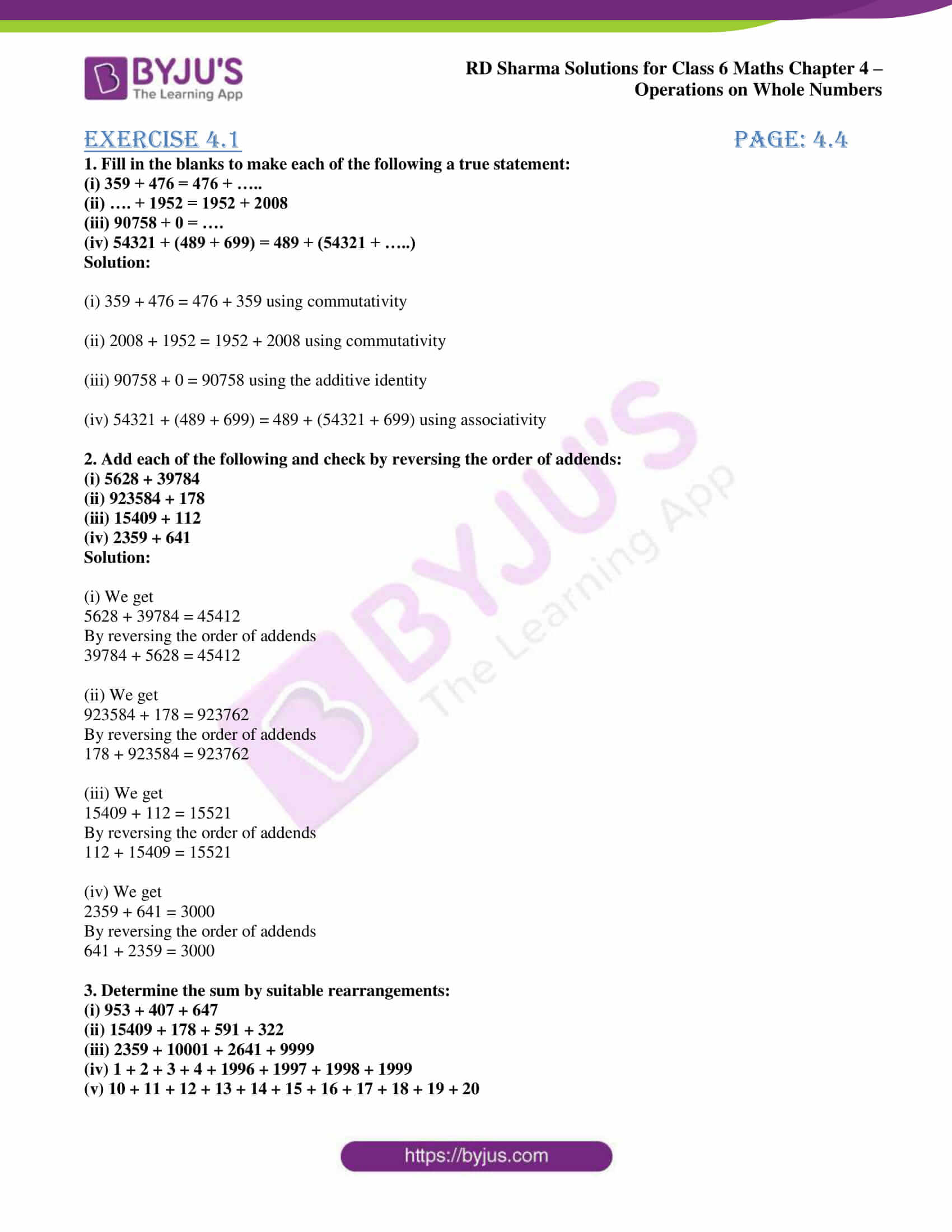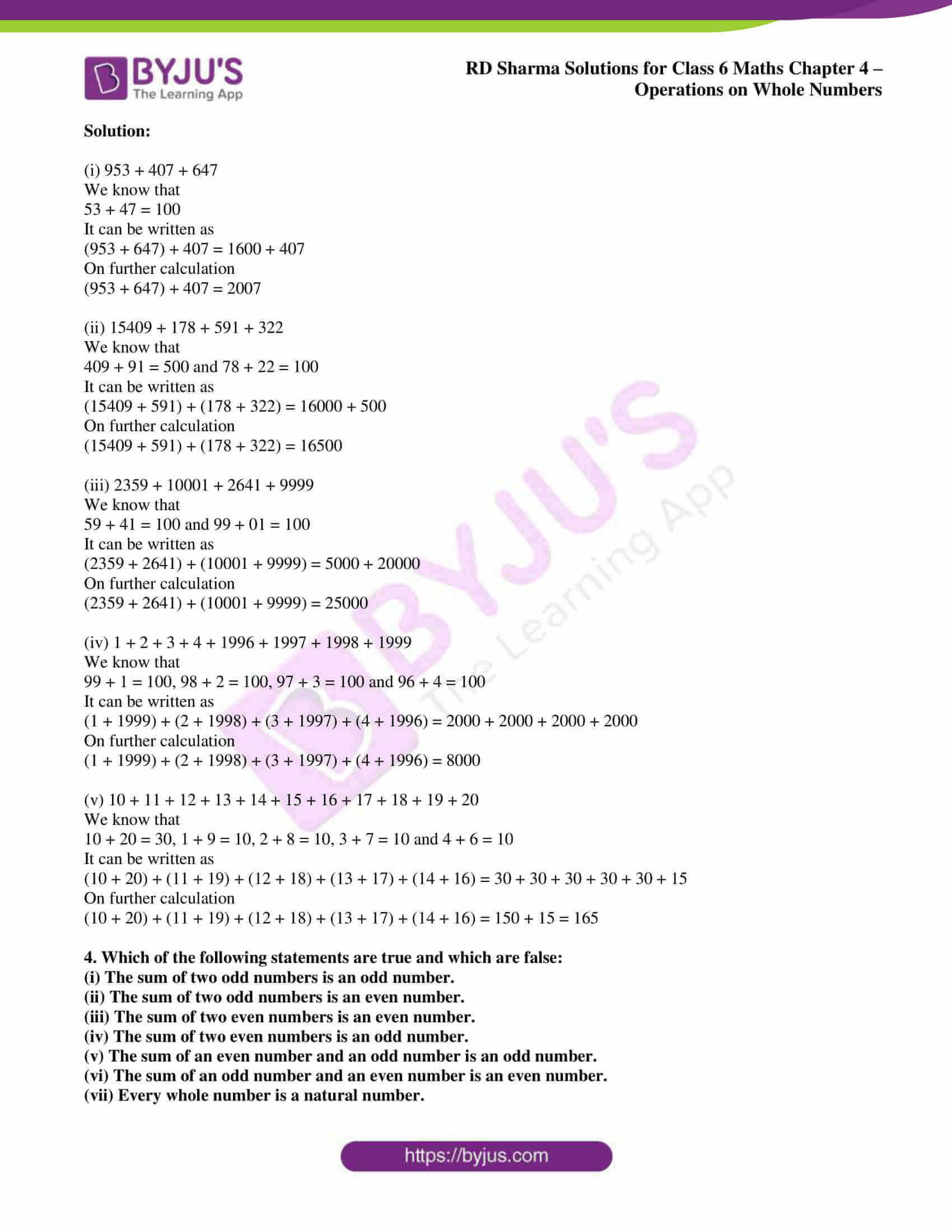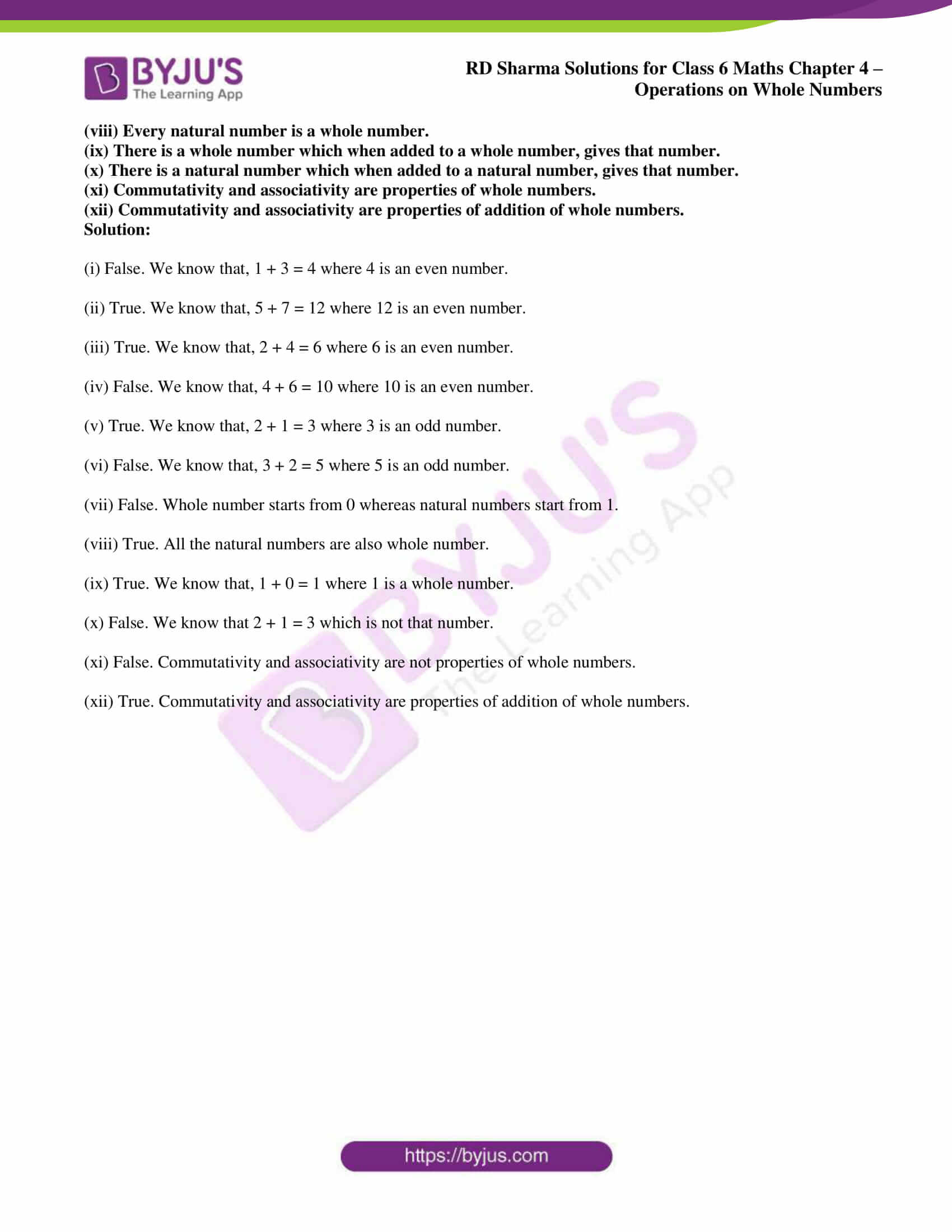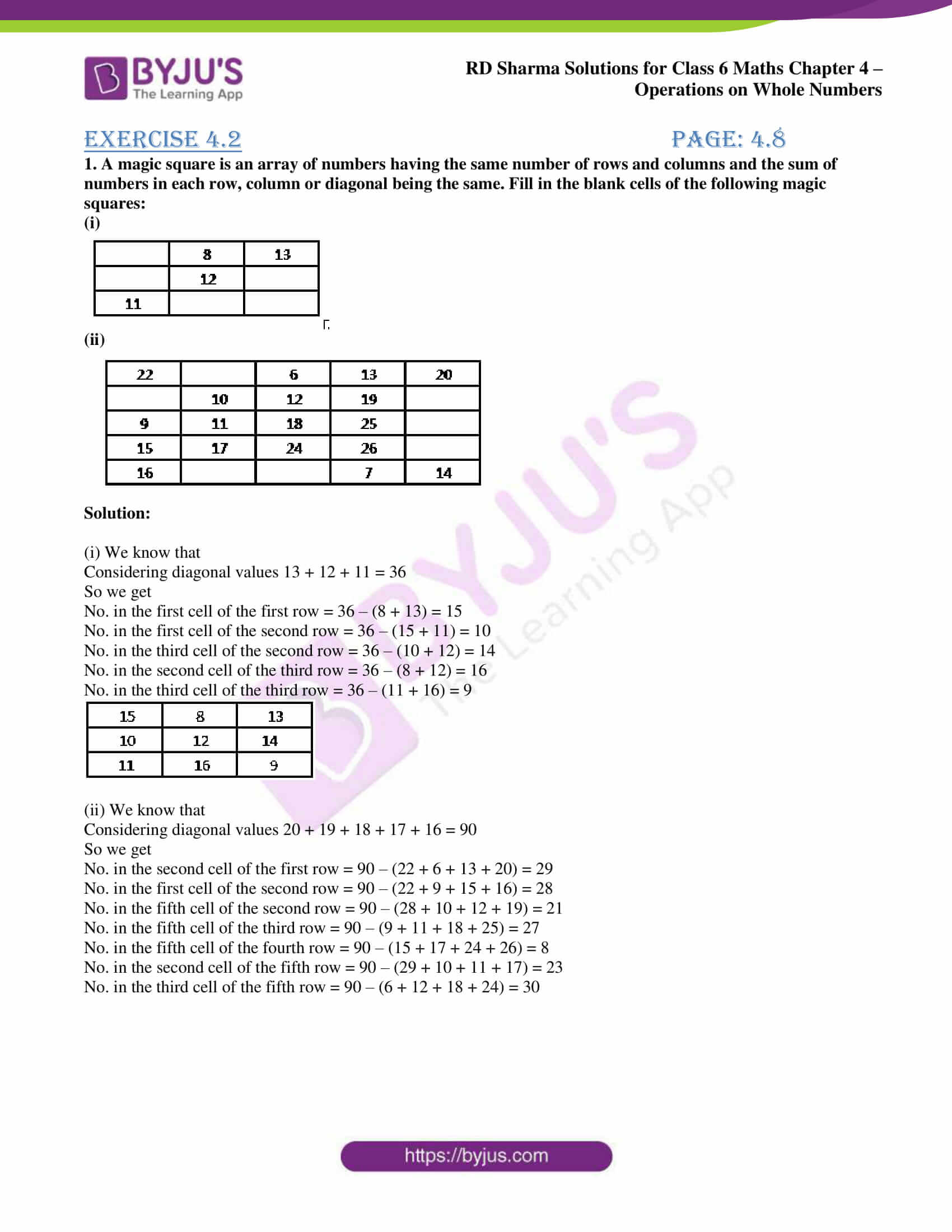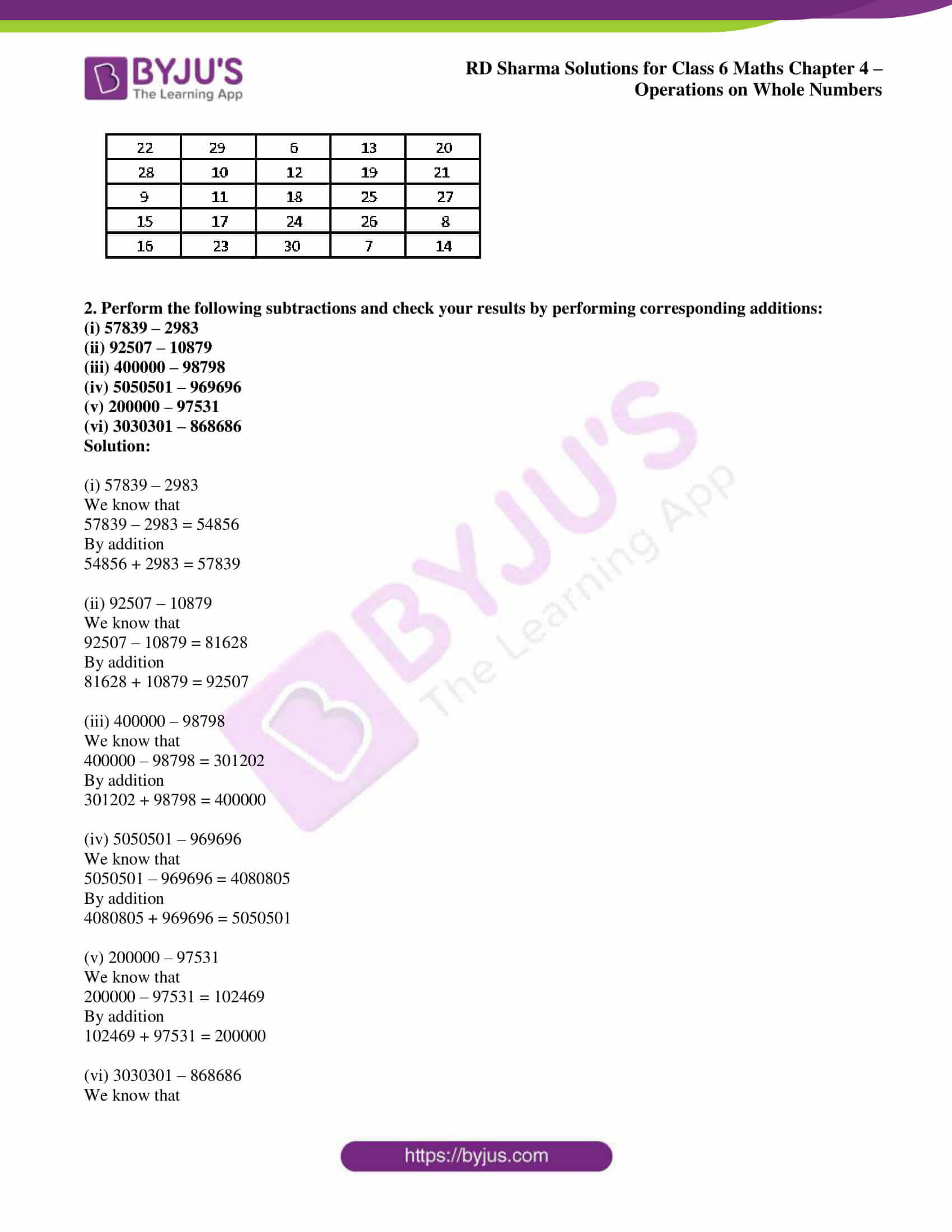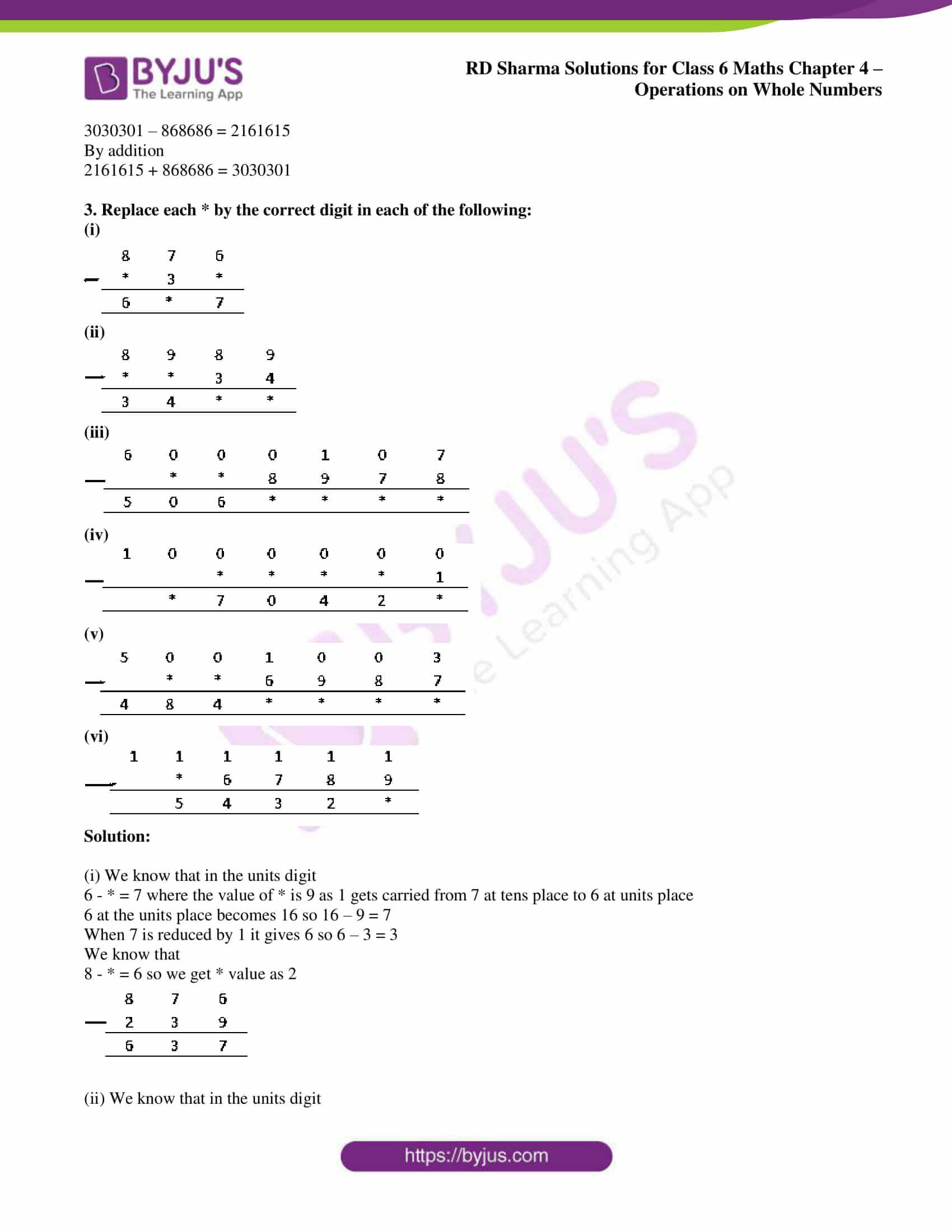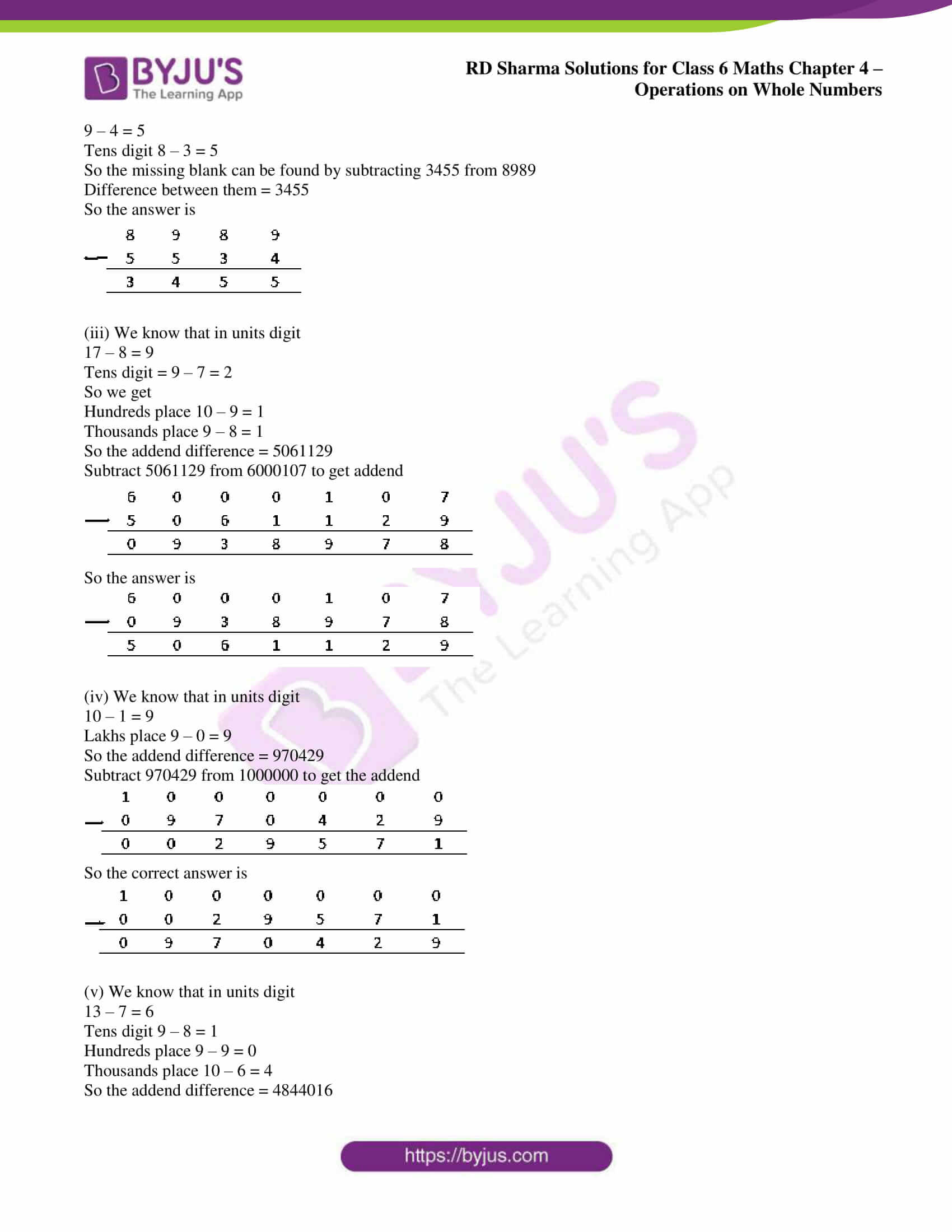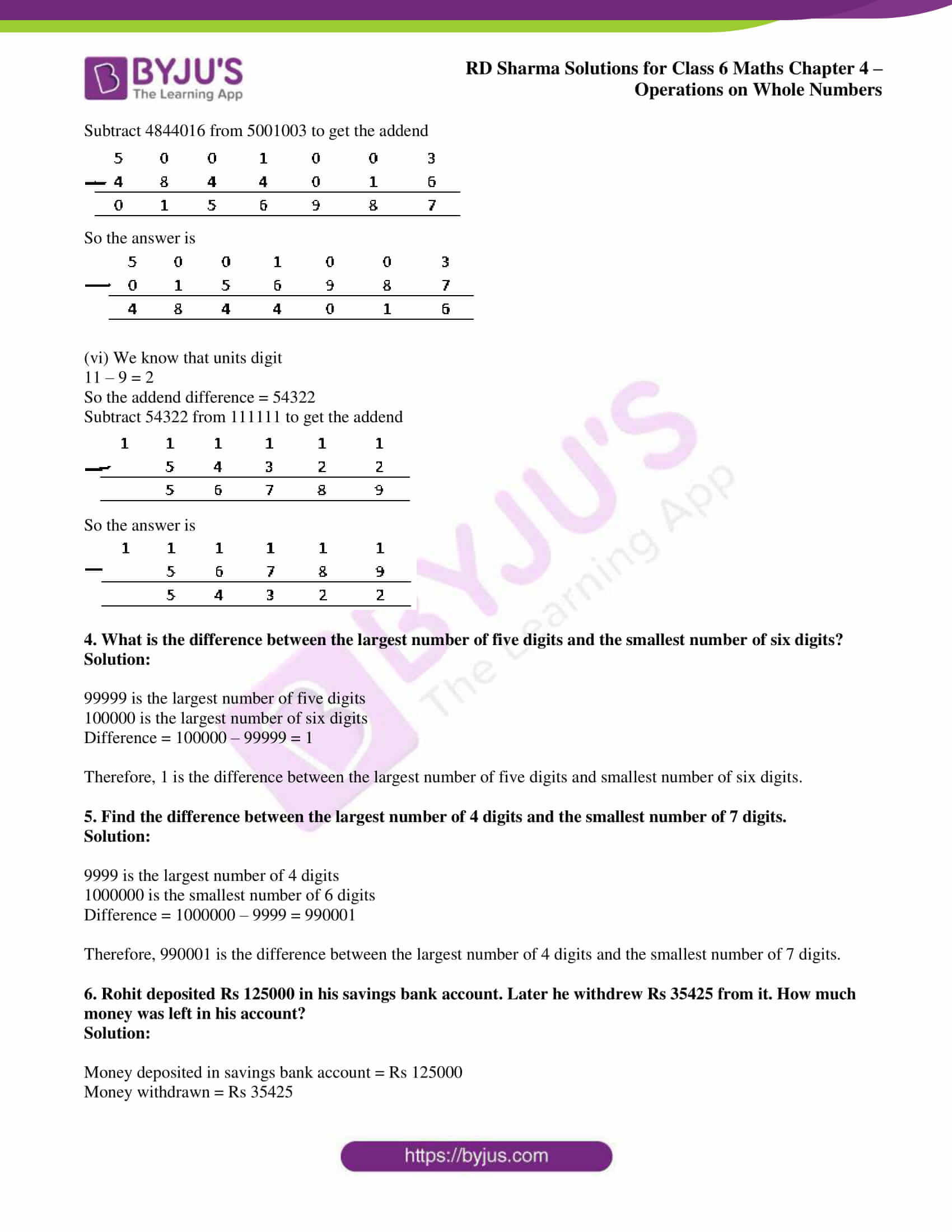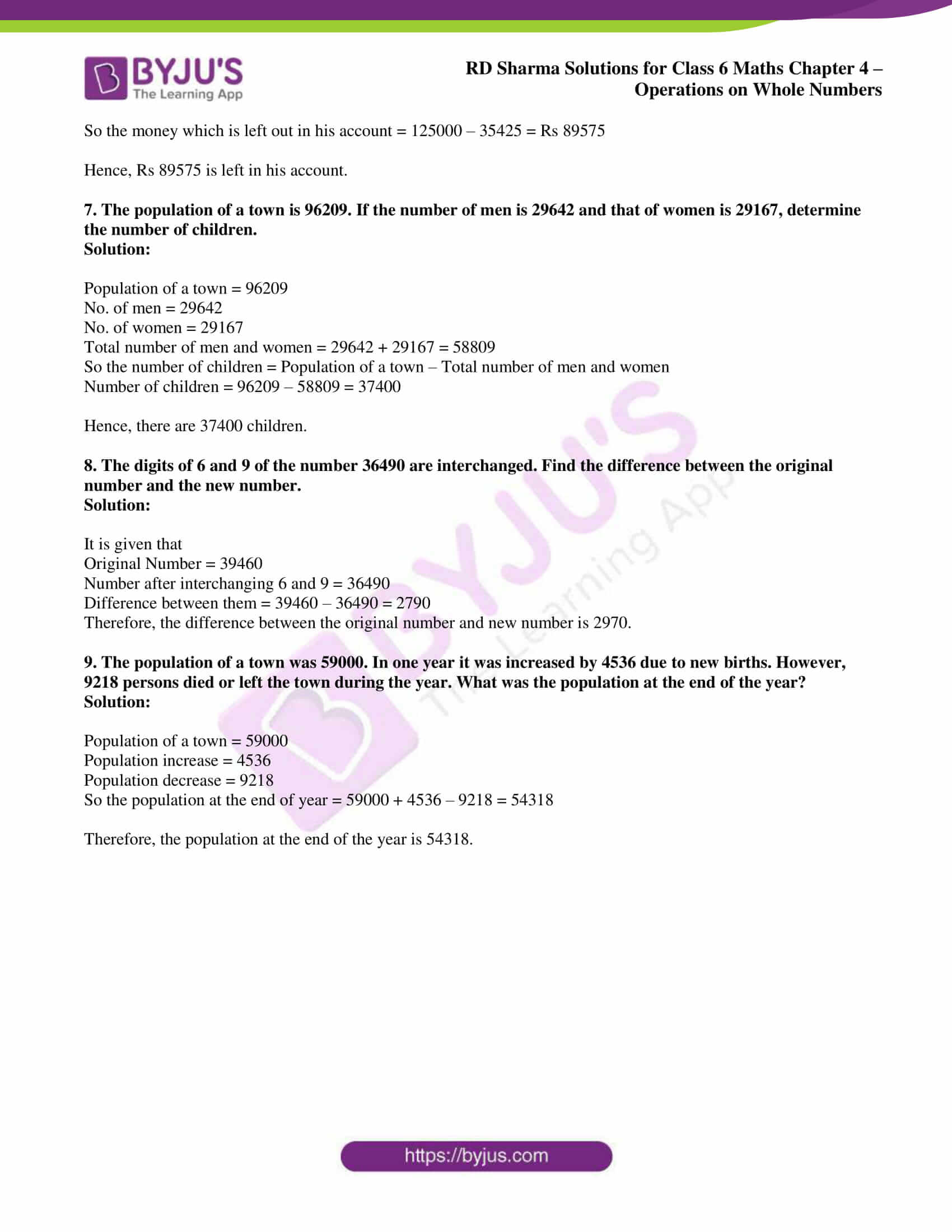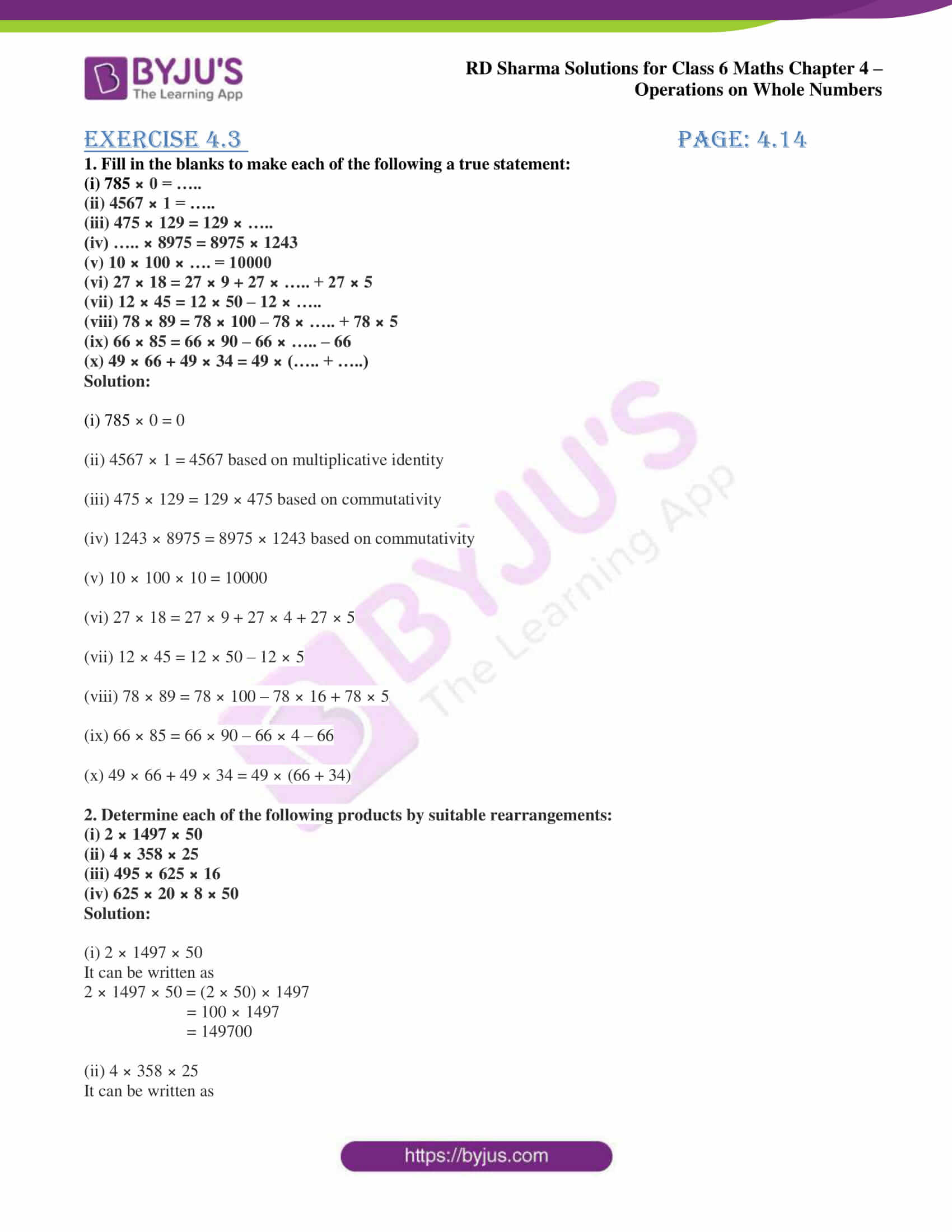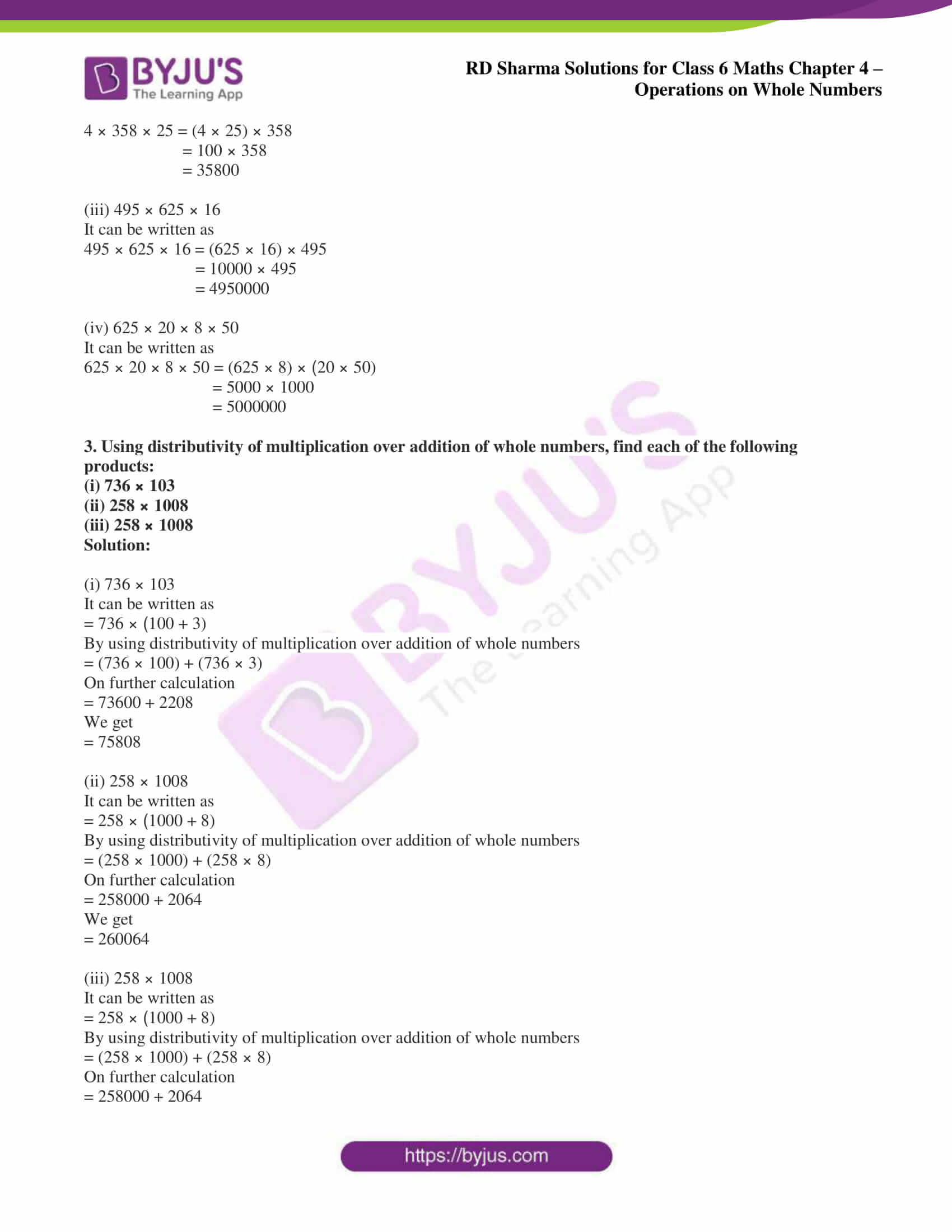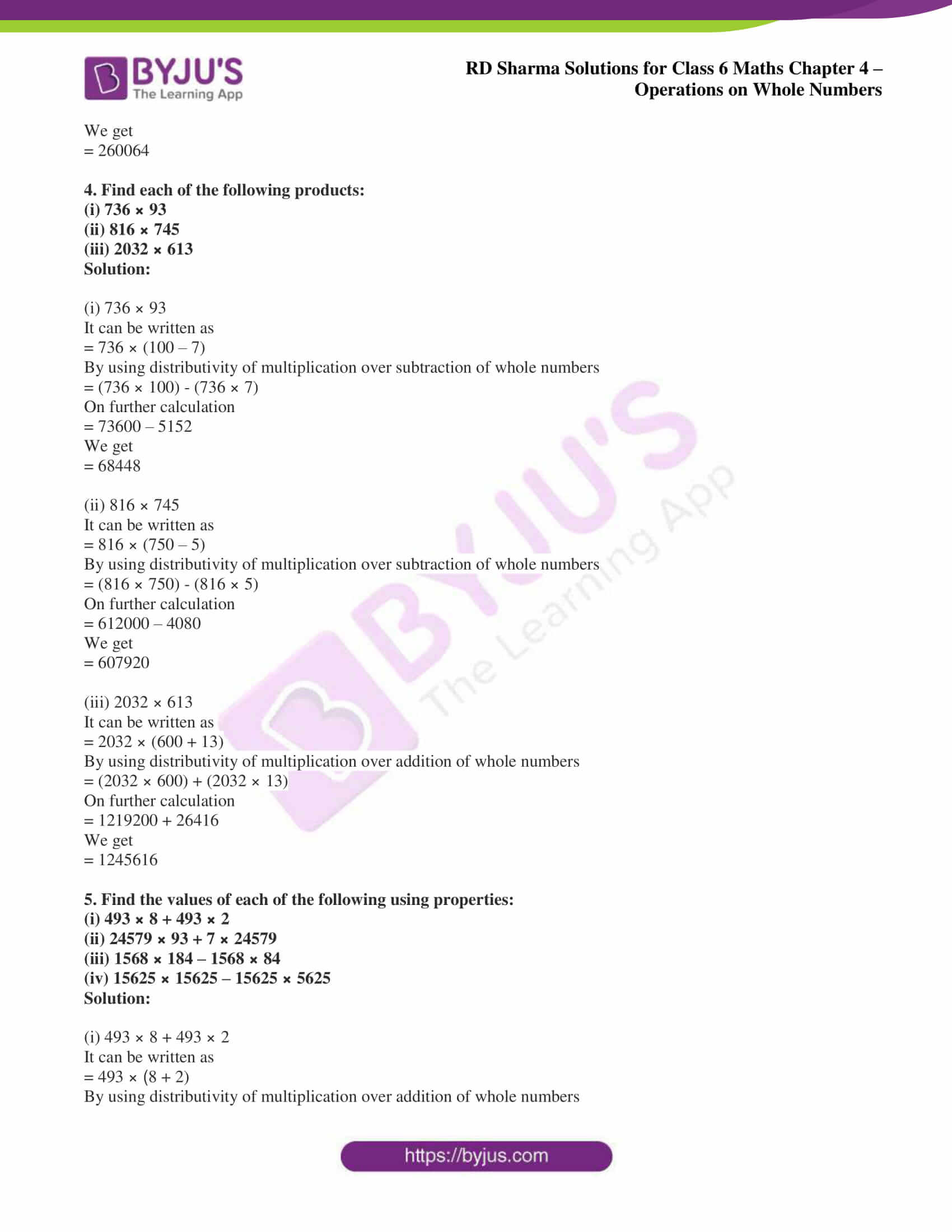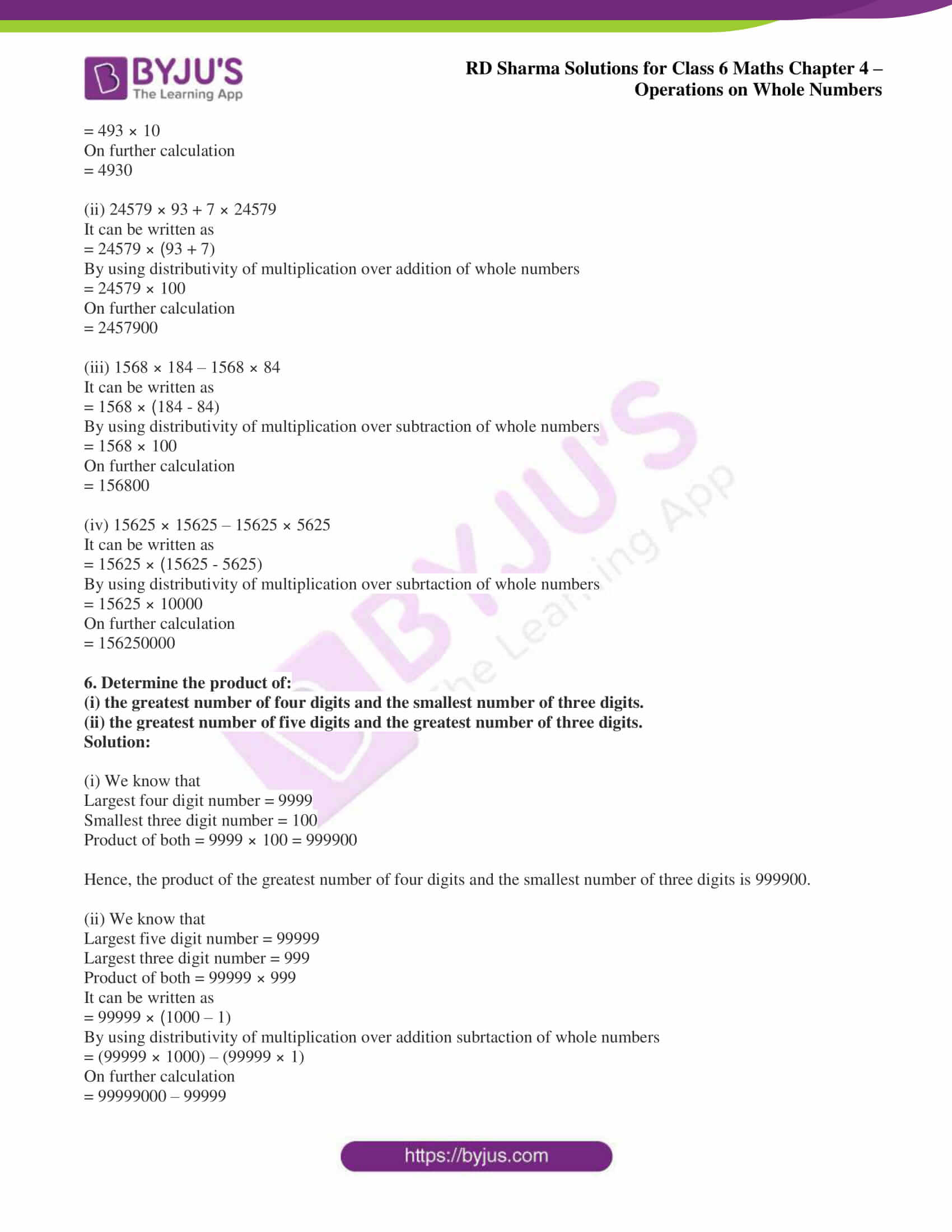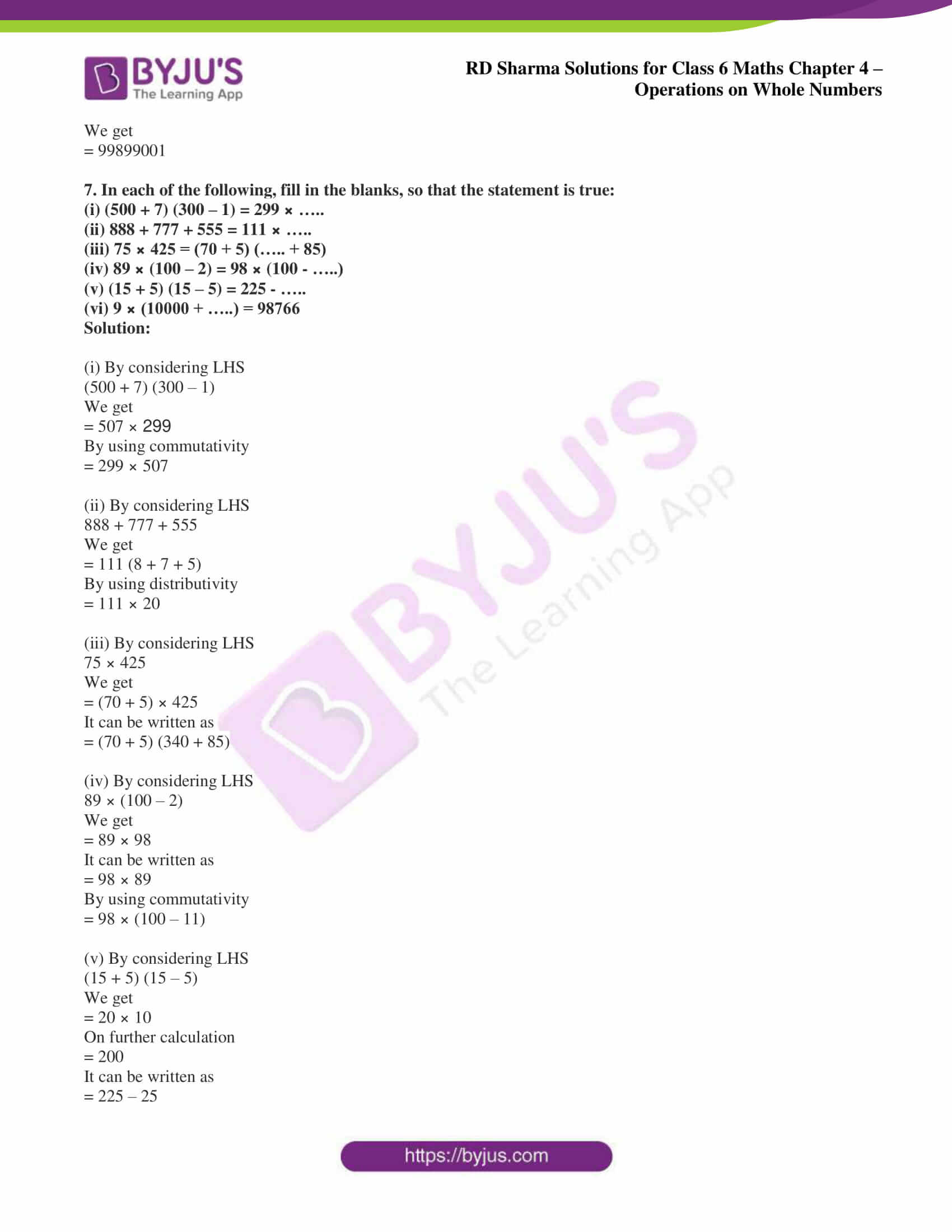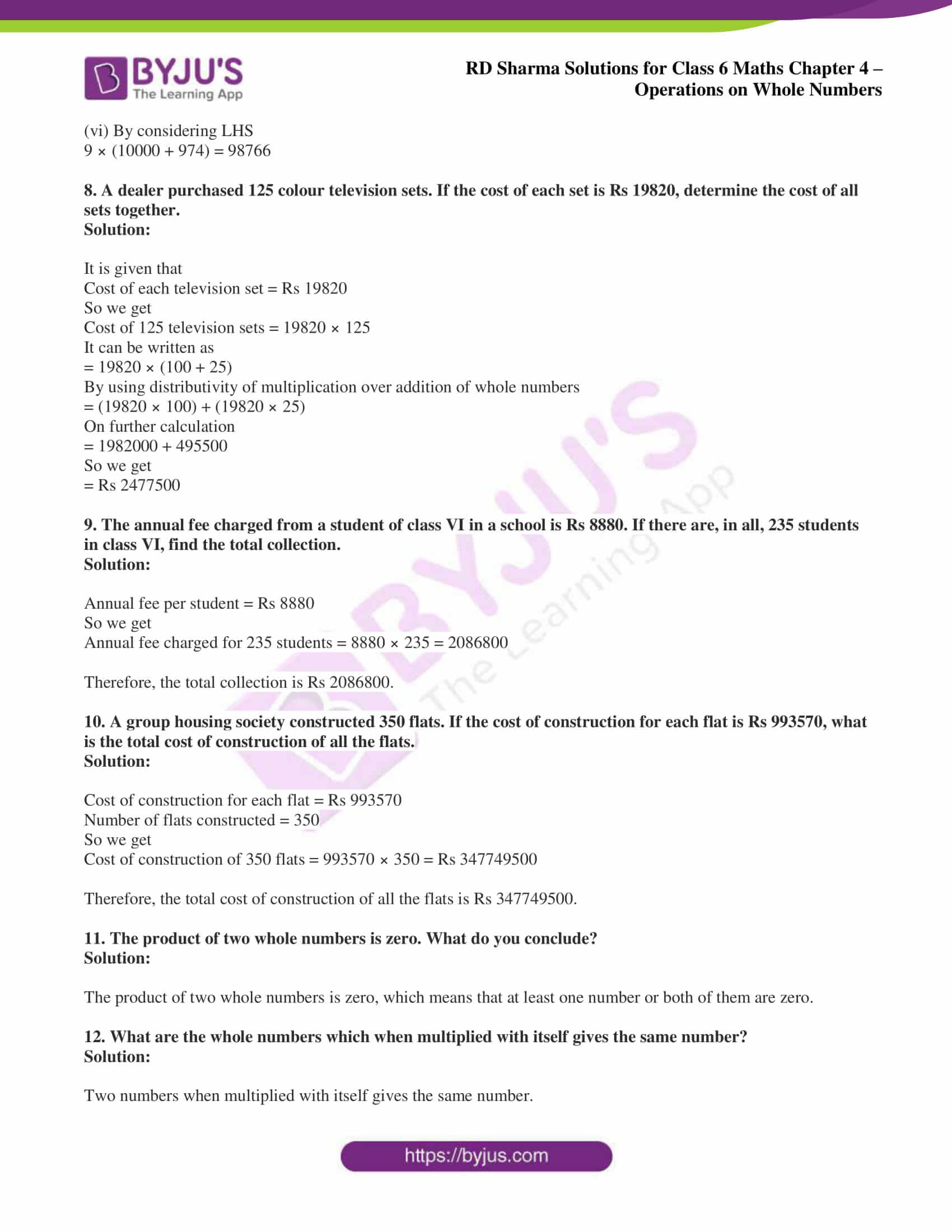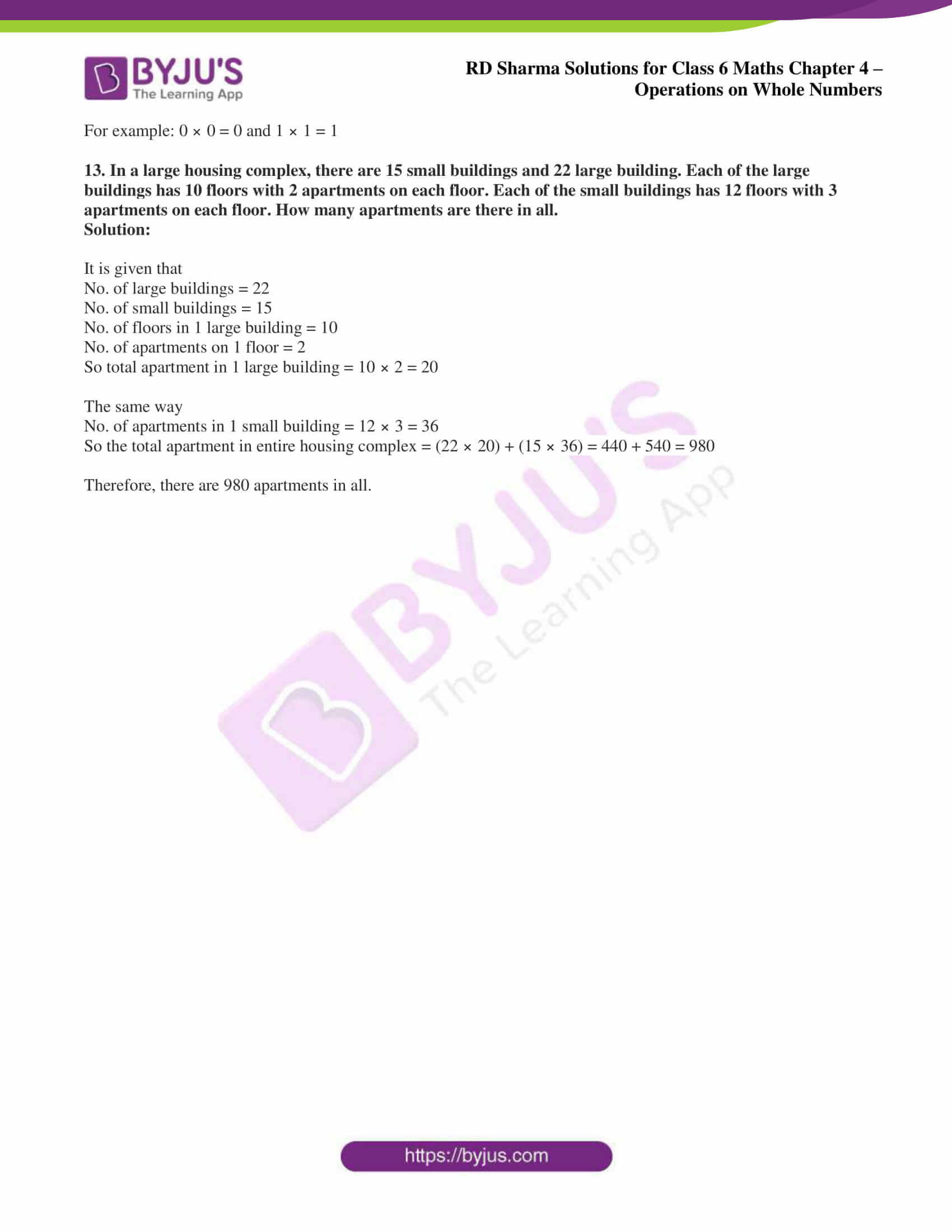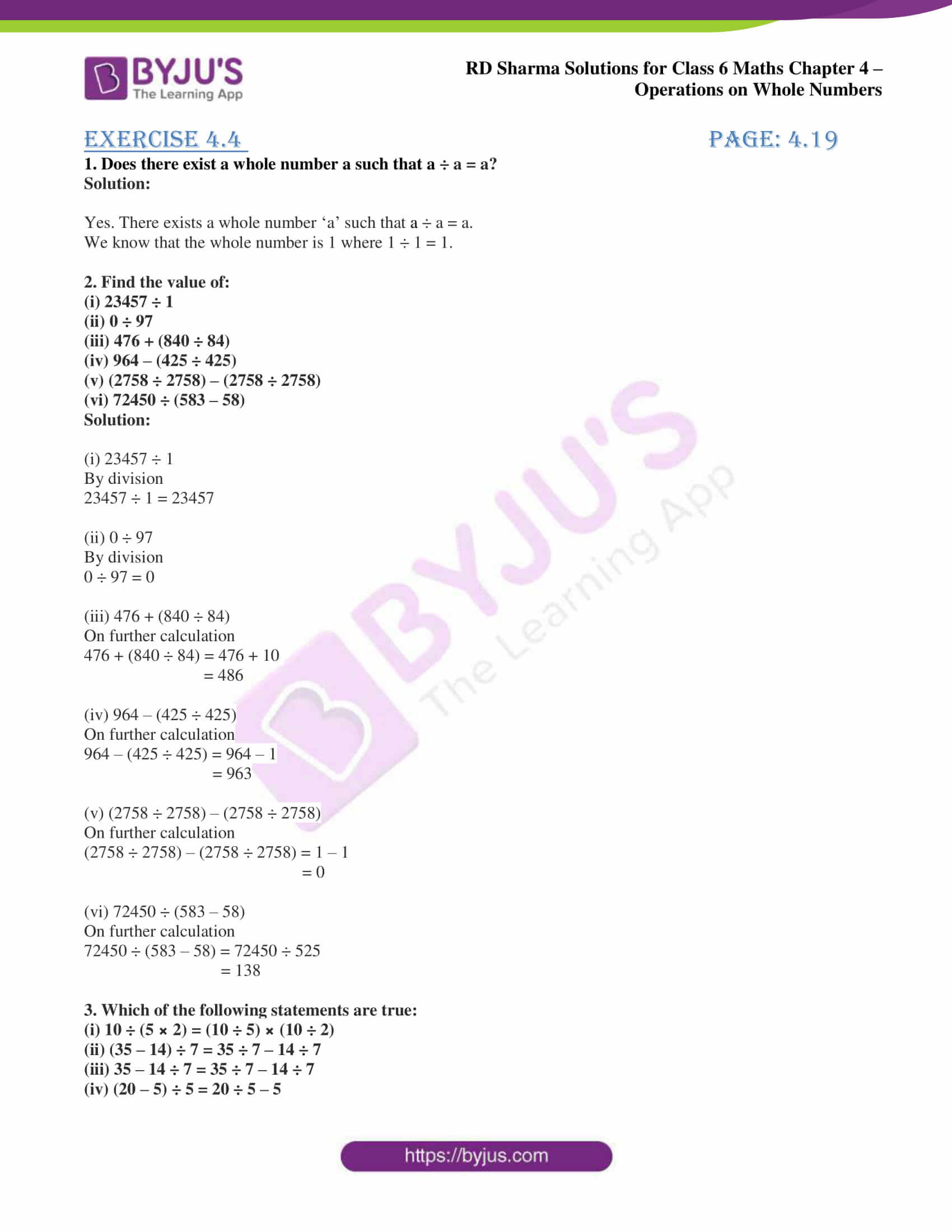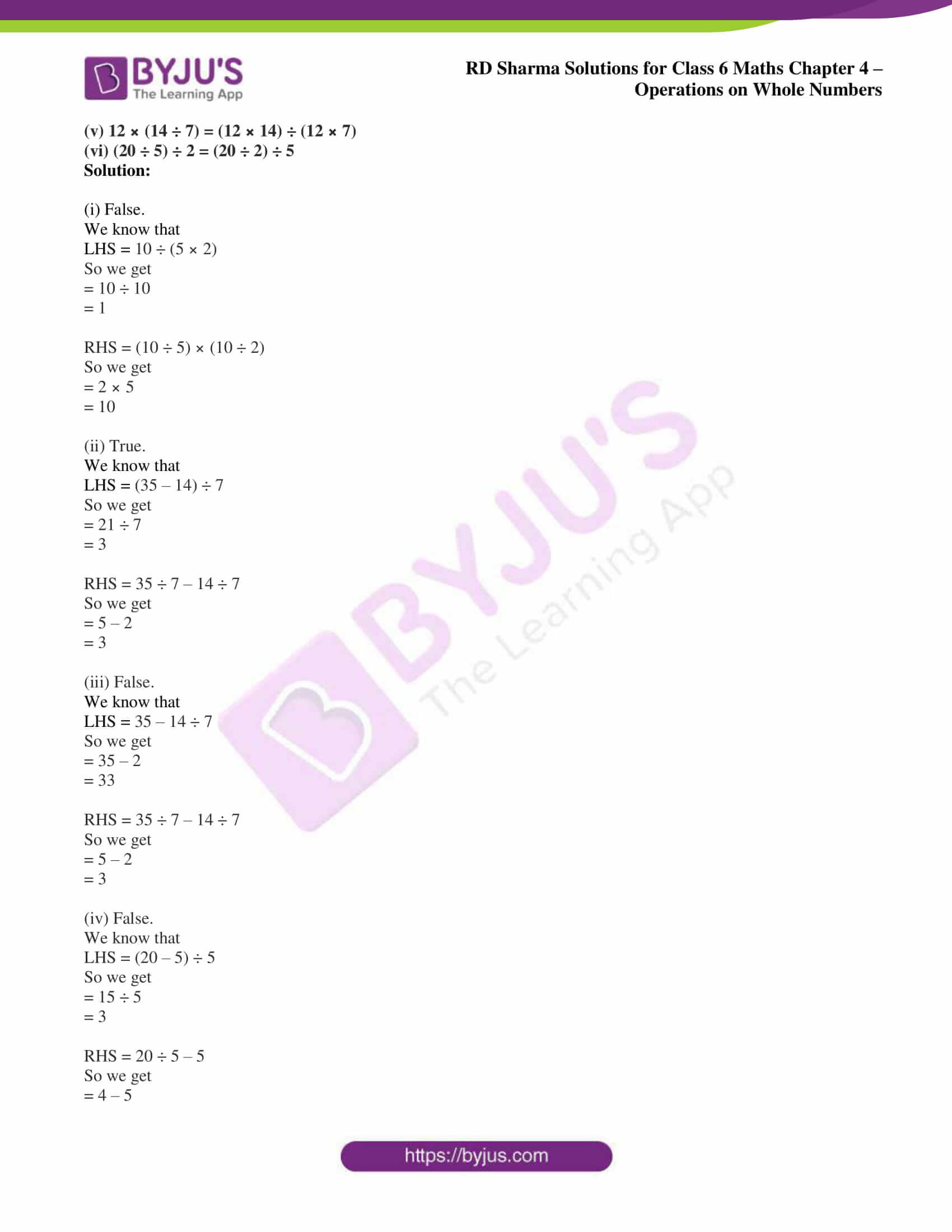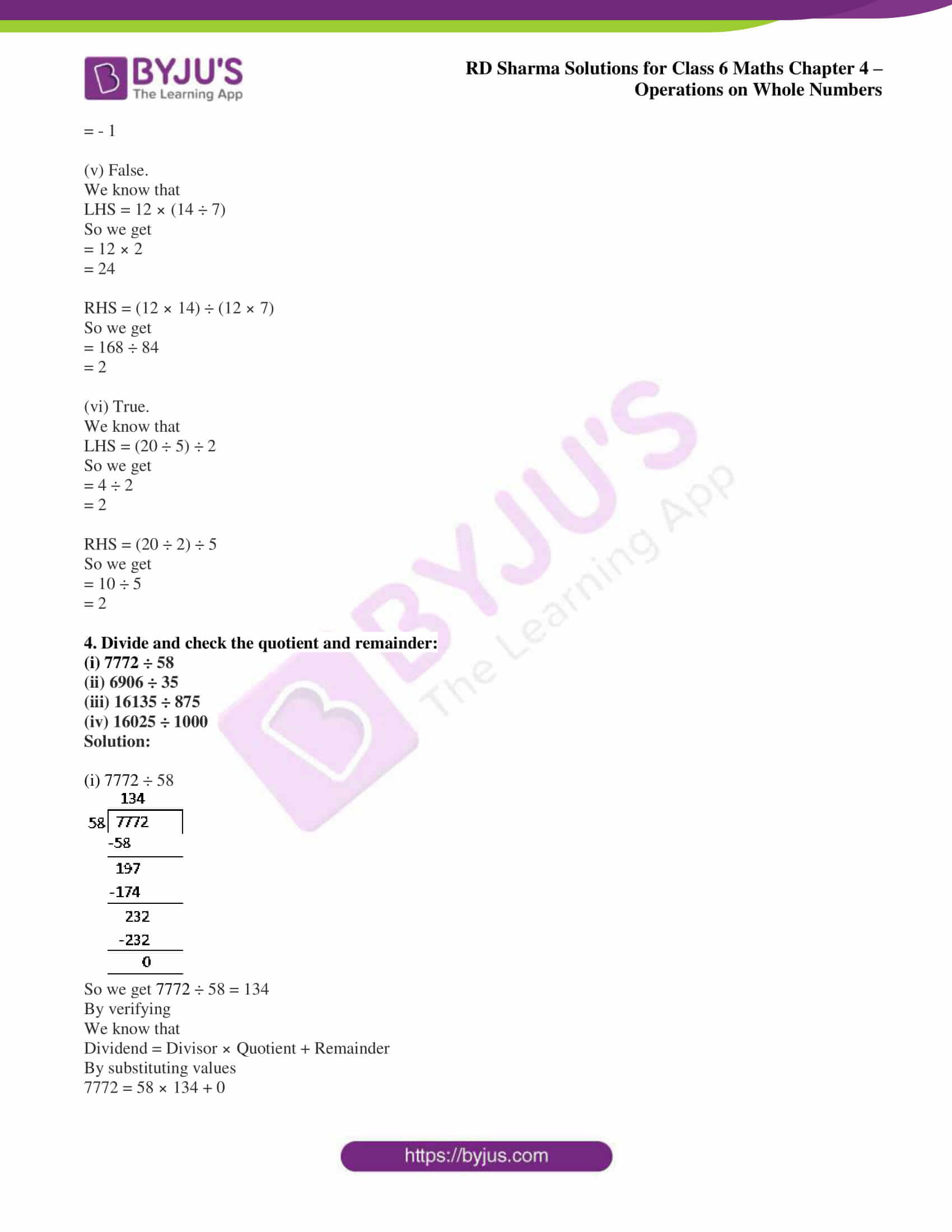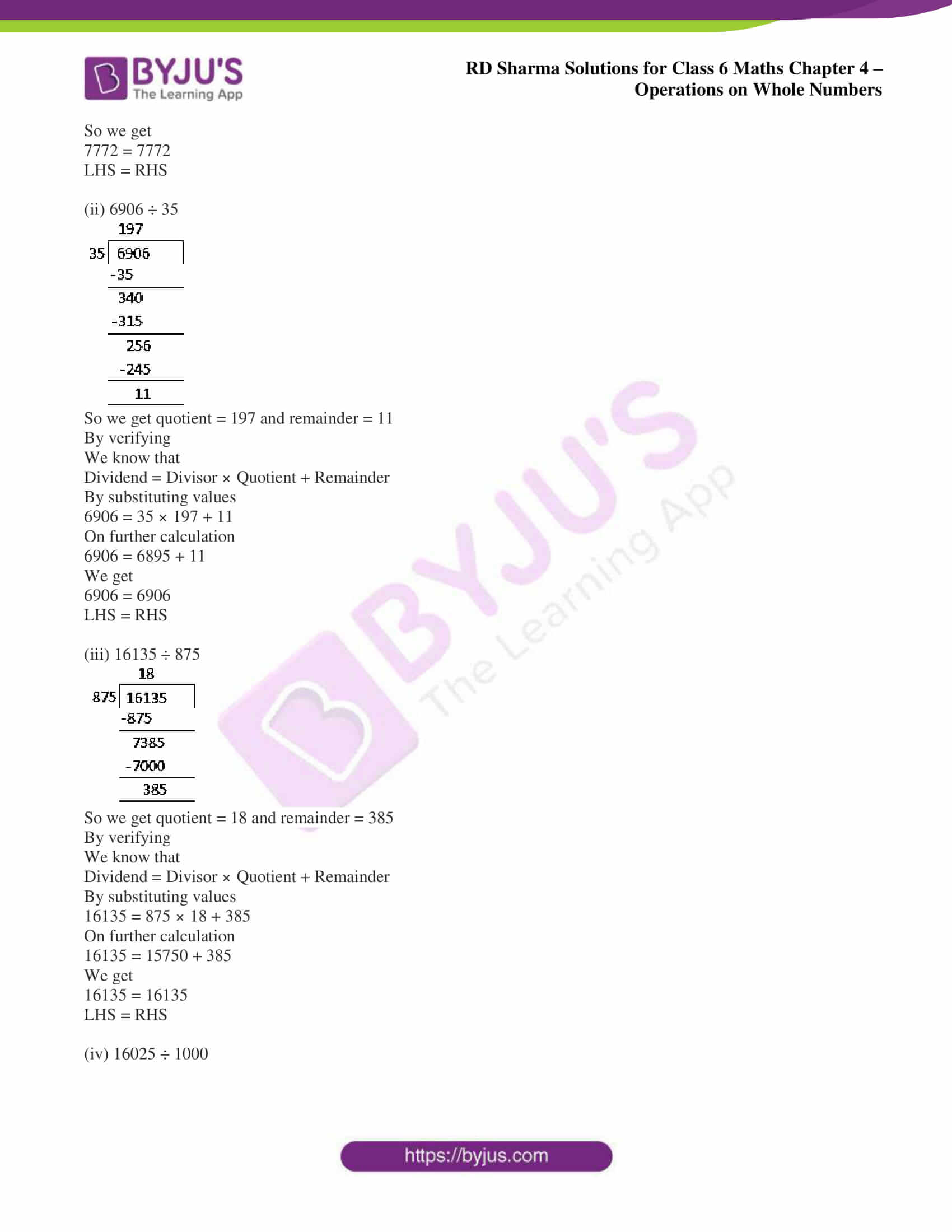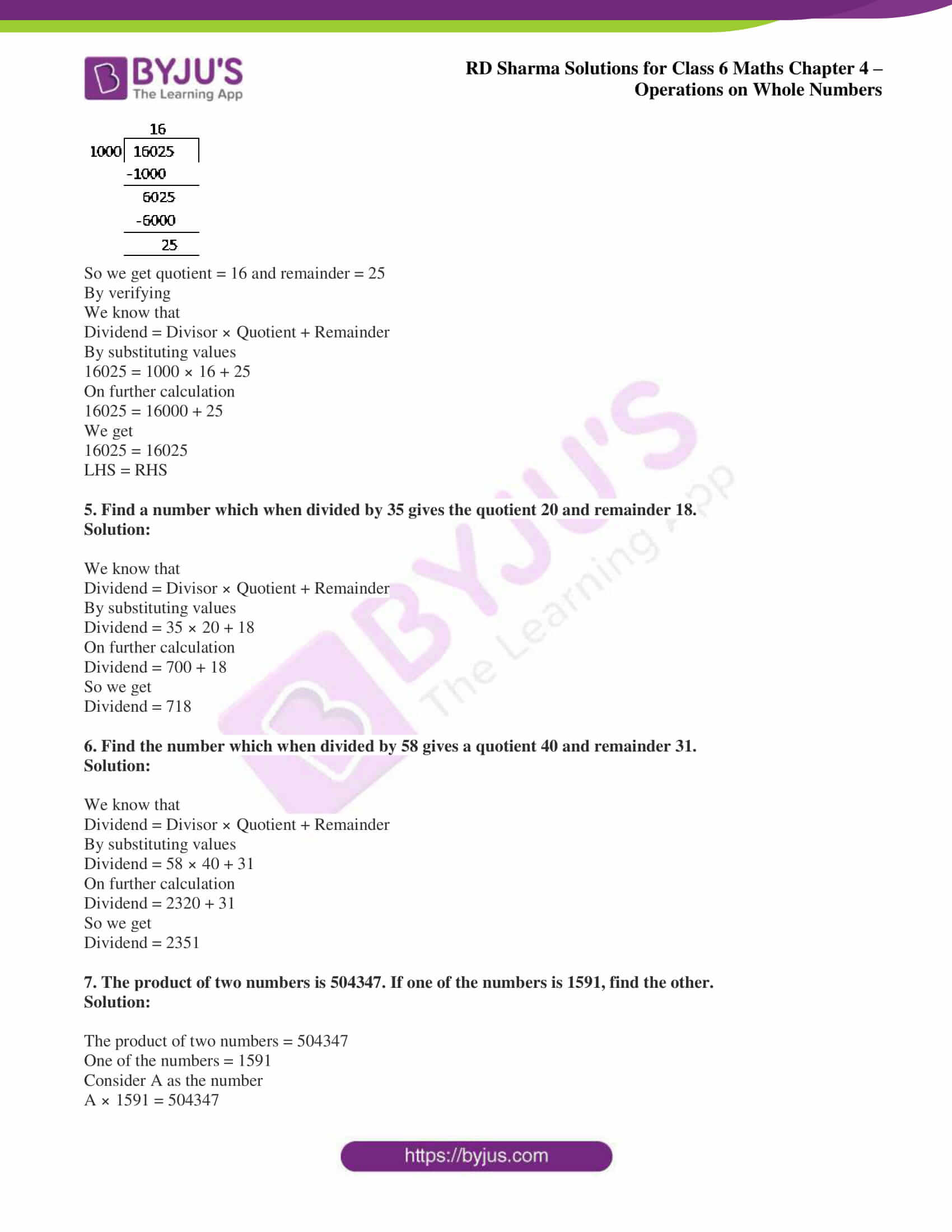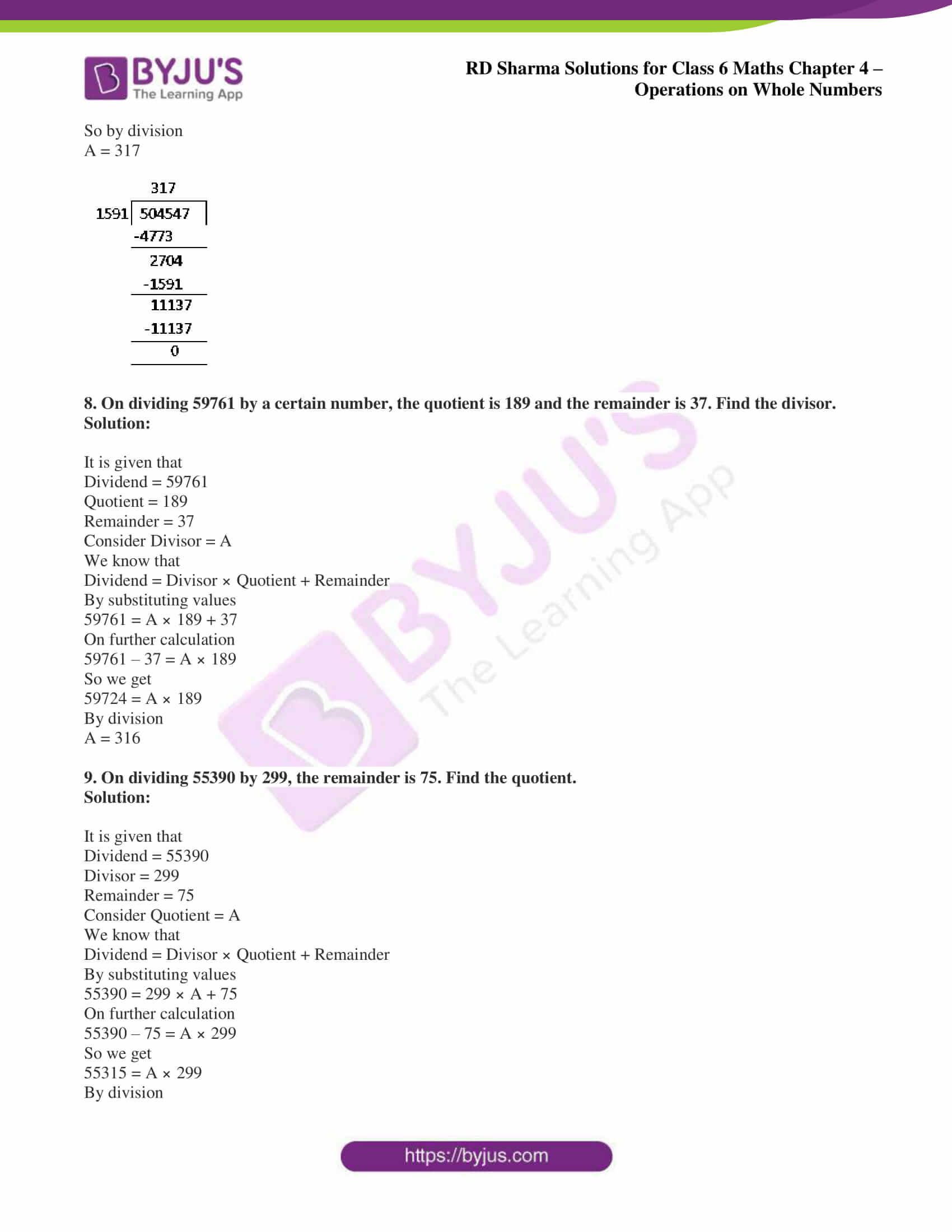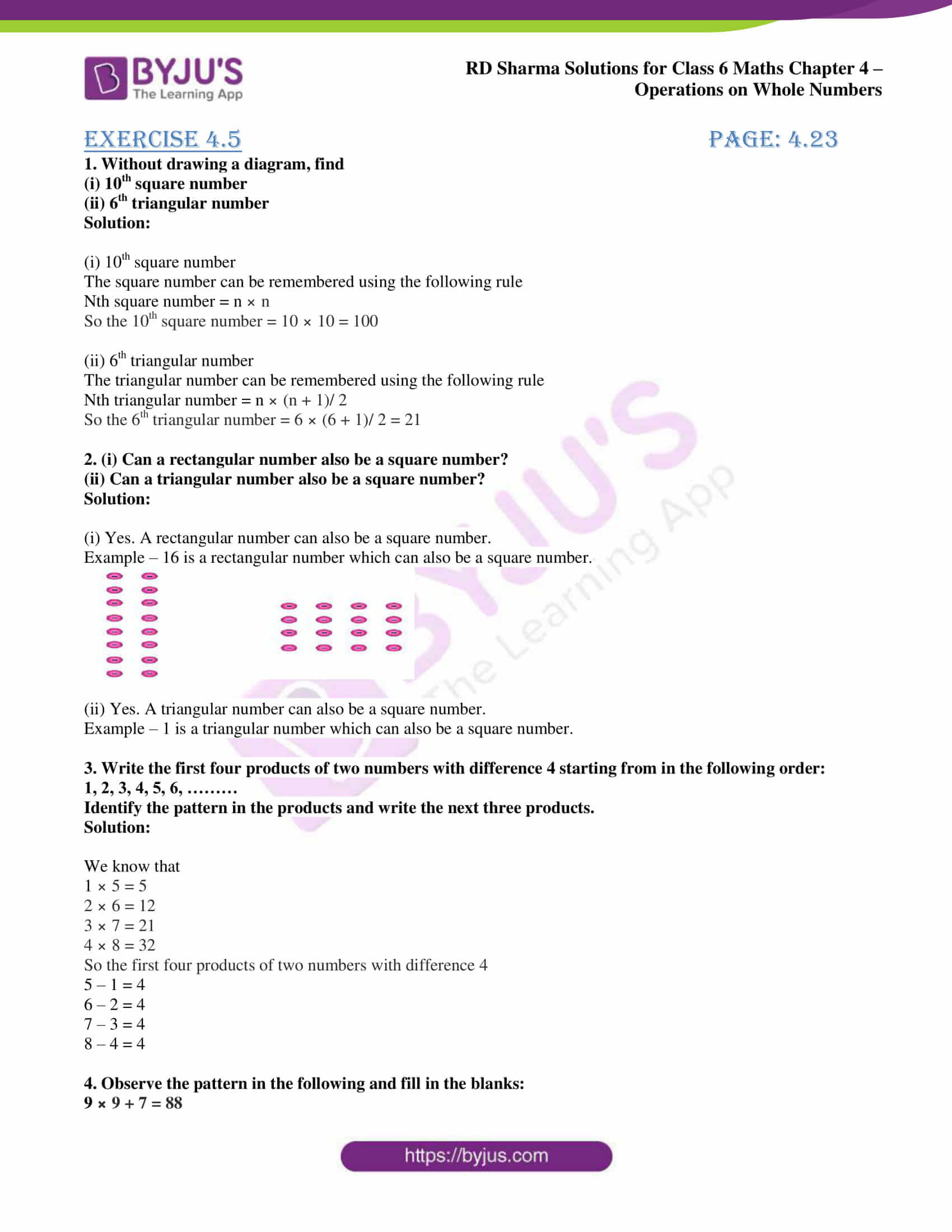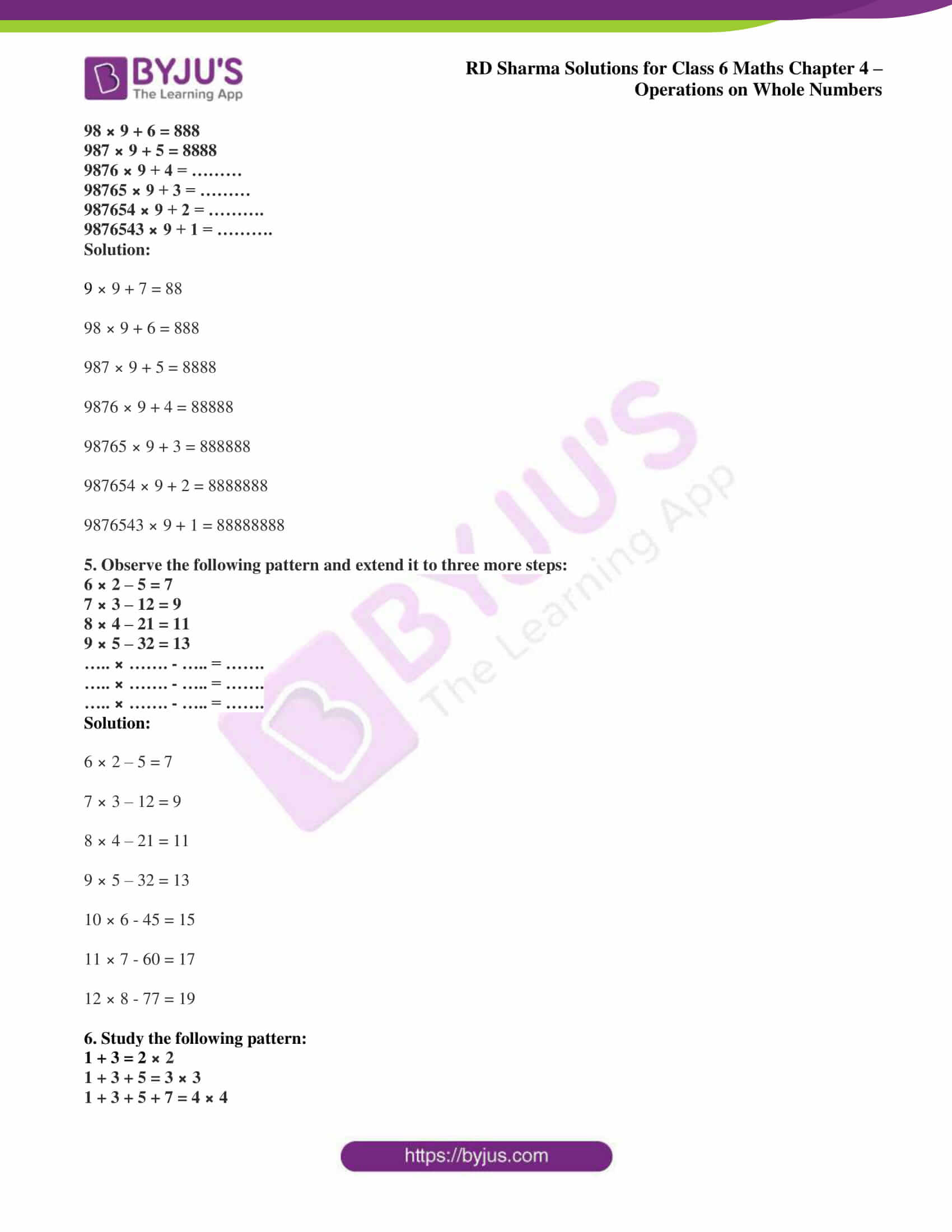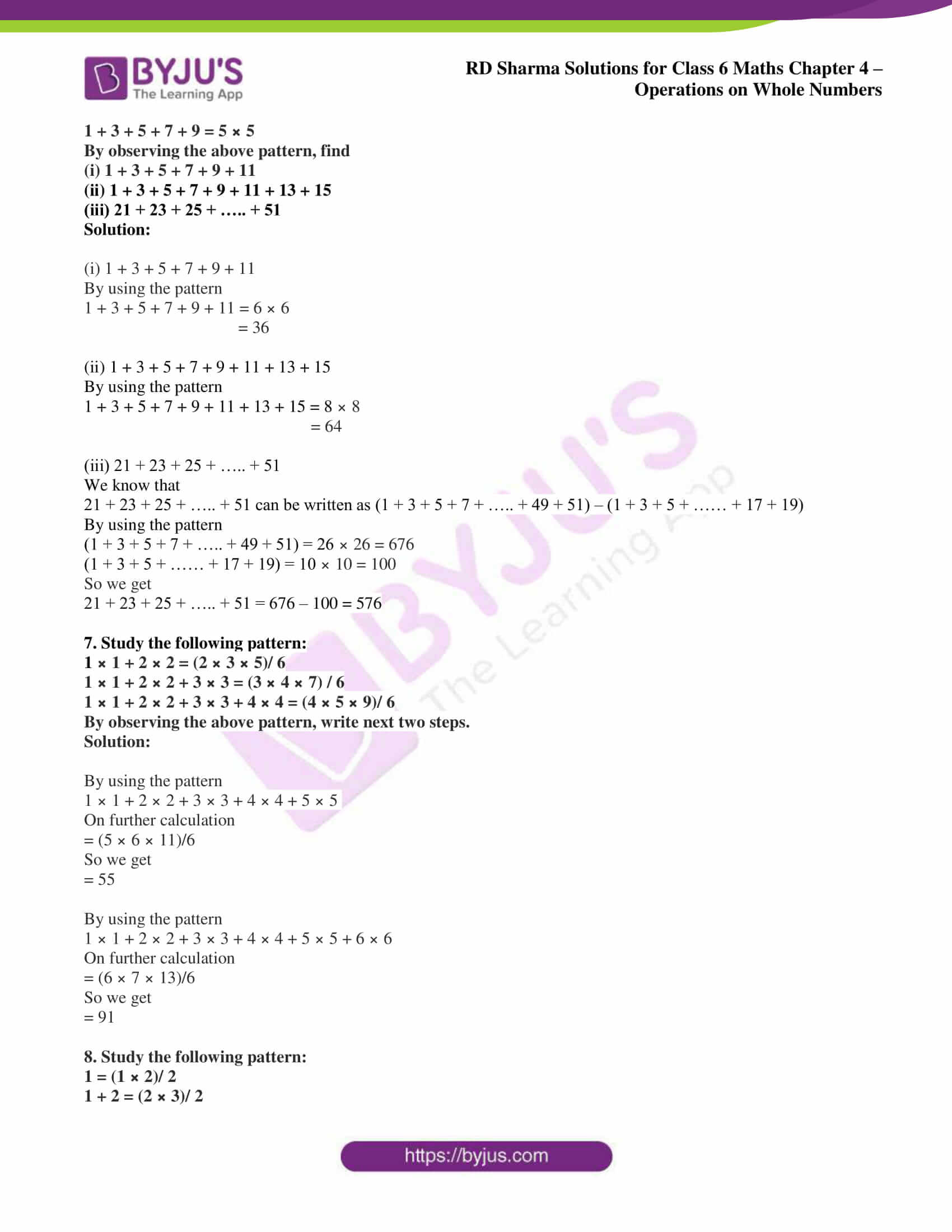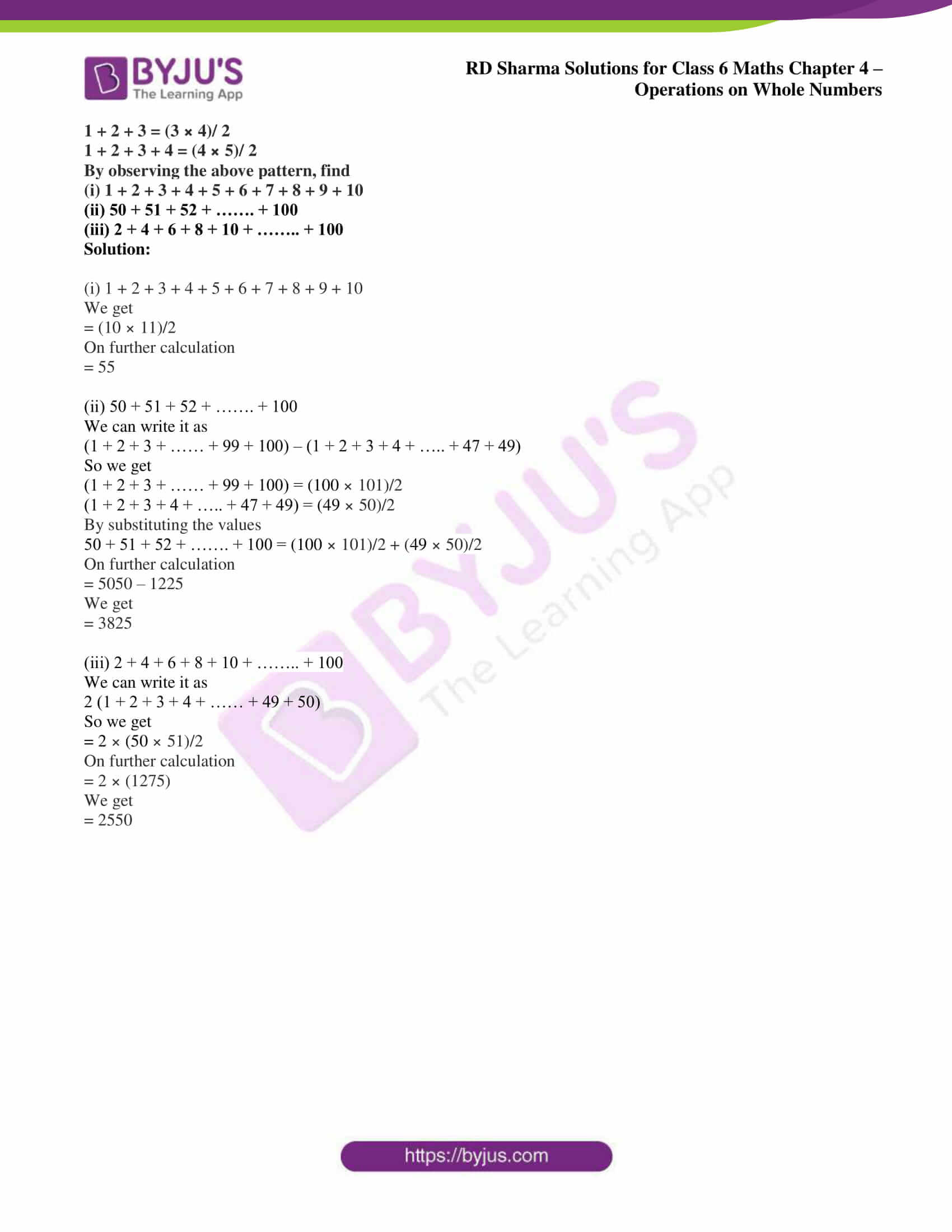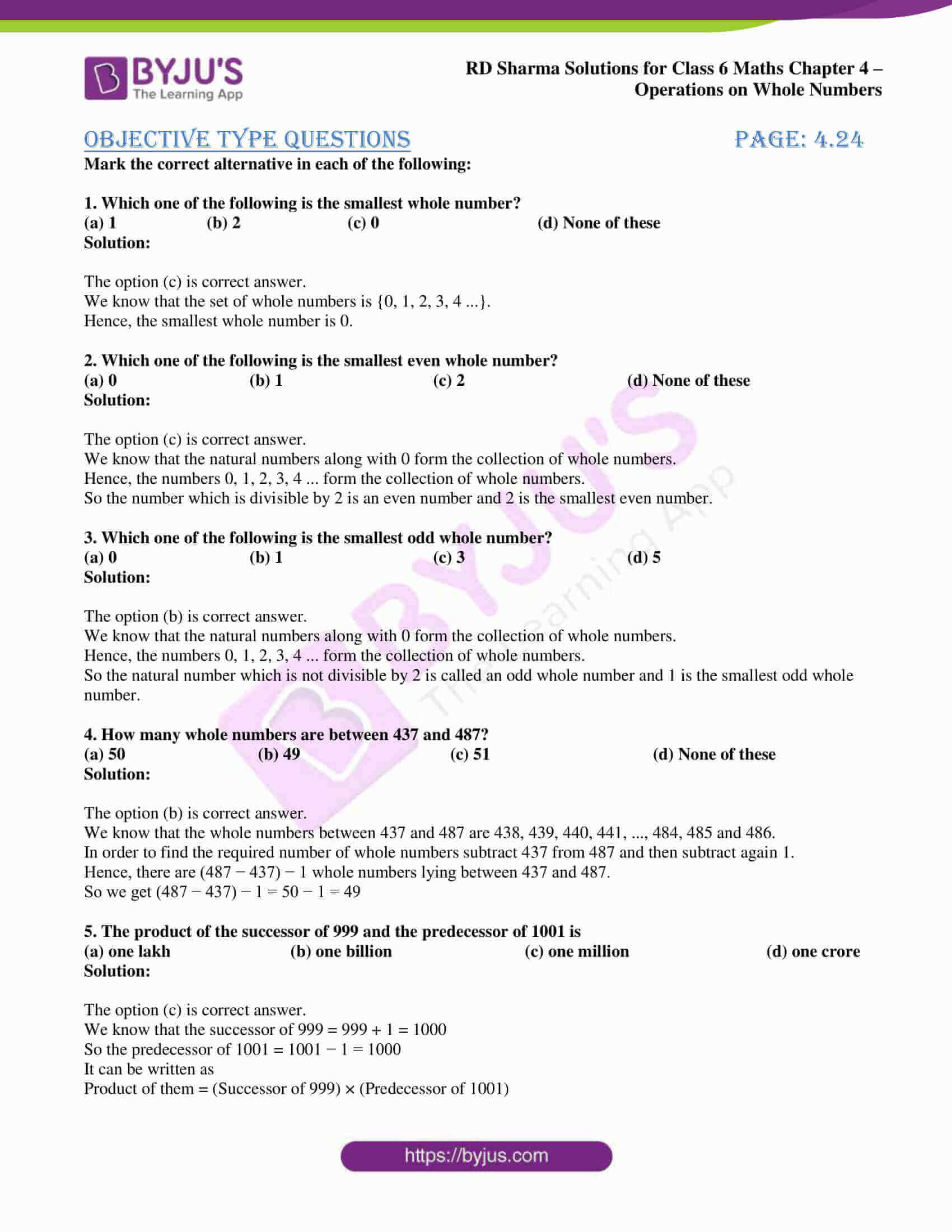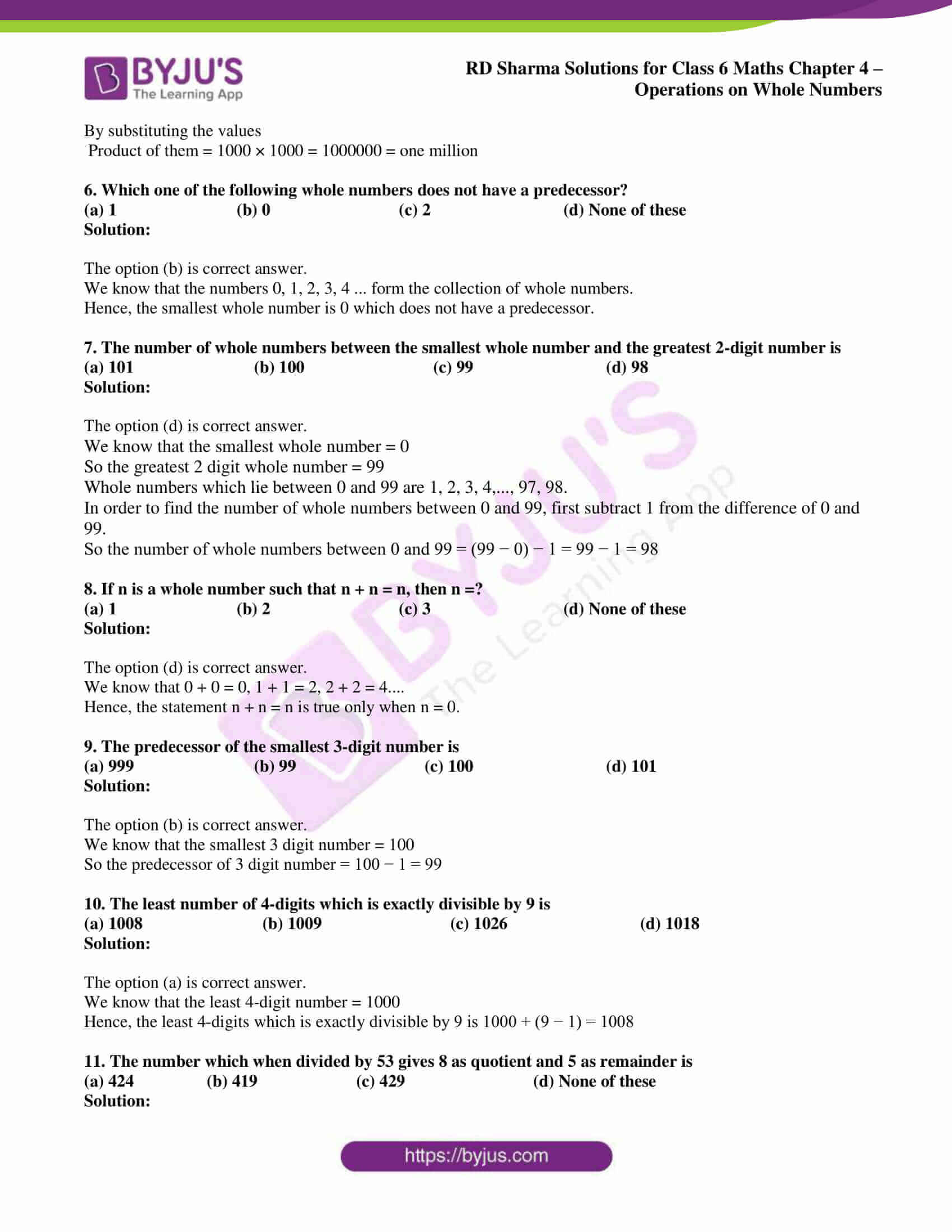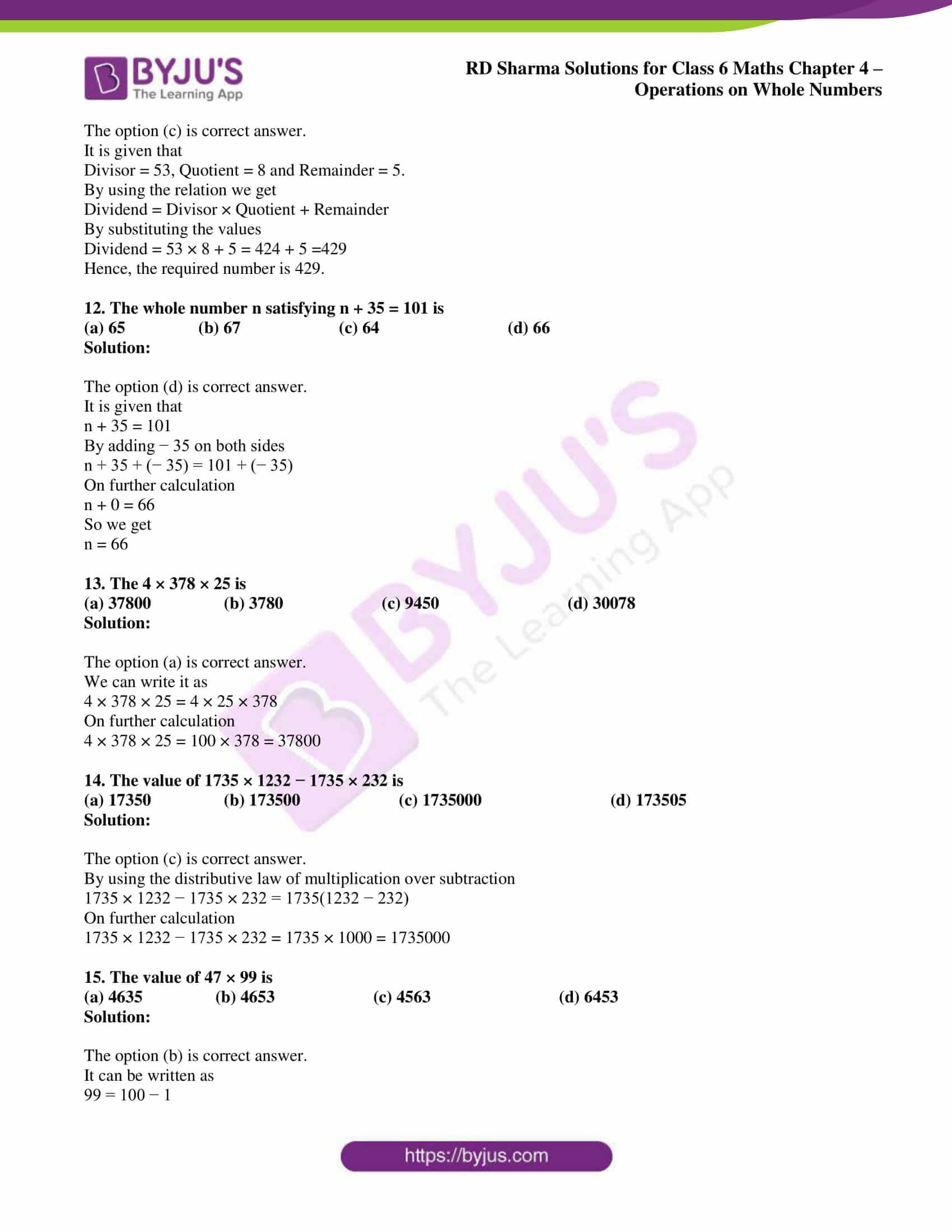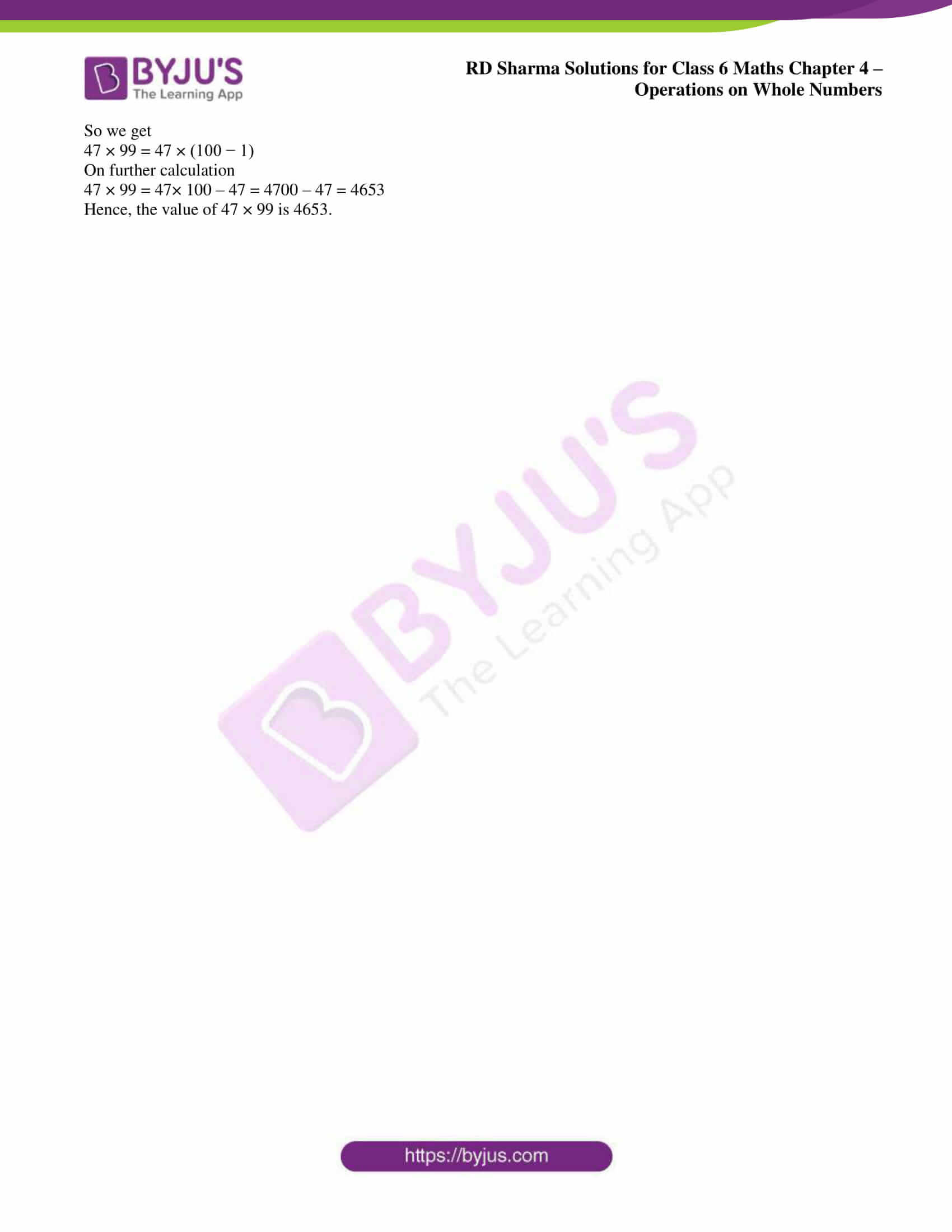## Access answers to Maths RD Sharma Solutions for Class 6 Chapter 4: Operations on Whole Numbers

### Exercise 4.1 PAGE: 4.4

1. Fill in the blanks to make each of the following a true statement:

(i) 359 + 476 = 476 + …..

(ii) …. + 1952 = 1952 + 2008

(iii) 90758 + 0 = ….

(iv) 54321 + (489 + 699) = 489 + (54321 + …..)

Solution:

(i) 359 + 476 = 476 + 359 using commutativity

(ii) 2008 + 1952 = 1952 + 2008 using commutativity

(iii) 90758 + 0 = 90758 using the additive identity

(iv) 54321 + (489 + 699) = 489 + (54321 + 699) using associativity

2. Add each of the following and check by reversing the order of addends:

(i) 5628 + 39784

(ii) 923584 + 178

(iii) 15409 + 112

(iv) 2359 + 641

Solution:

(i) We get

5628 + 39784 = 45412

By reversing the order of addends

39784 + 5628 = 45412

(ii) We get

923584 + 178 = 923762

By reversing the order of addends

178 + 923584 = 923762

(iii) We get

15409 + 112 = 15521

By reversing the order of addends

112 + 15409 = 15521

(iv) We get

2359 + 641 = 3000

By reversing the order of addends

641 + 2359 = 3000

3. Determine the sum by suitable rearrangements:

(i) 953 + 407 + 647

(ii) 15409 + 178 + 591 + 322

(iii) 2359 + 10001 + 2641 + 9999

(iv) 1 + 2 + 3 + 4 + 1996 + 1997 + 1998 + 1999

(v) 10 + 11 + 12 + 13 + 14 + 15 + 16 + 17 + 18 + 19 + 20

Solution:

(i) 953 + 407 + 647

We know that

53 + 47 = 100

It can be written as

(953 + 647) + 407 = 1600 + 407

On further calculation

(953 + 647) + 407 = 2007

(ii) 15409 + 178 + 591 + 322

We know that

409 + 91 = 500 and 78 + 22 = 100

It can be written as

(15409 + 591) + (178 + 322) = 16000 + 500

On further calculation

(15409 + 591) + (178 + 322) = 16500

(iii) 2359 + 10001 + 2641 + 9999

We know that

59 + 41 = 100 and 99 + 01 = 100

It can be written as

(2359 + 2641) + (10001 + 9999) = 5000 + 20000

On further calculation

(2359 + 2641) + (10001 + 9999) = 25000

(iv) 1 + 2 + 3 + 4 + 1996 + 1997 + 1998 + 1999

We know that

99 + 1 = 100, 98 + 2 = 100, 97 + 3 = 100 and 96 + 4 = 100

It can be written as

(1 + 1999) + (2 + 1998) + (3 + 1997) + (4 + 1996) = 2000 + 2000 + 2000 + 2000

On further calculation

(1 + 1999) + (2 + 1998) + (3 + 1997) + (4 + 1996) = 8000

(v) 10 + 11 + 12 + 13 + 14 + 15 + 16 + 17 + 18 + 19 + 20

We know that

10 + 20 = 30, 1 + 9 = 10, 2 + 8 = 10, 3 + 7 = 10 and 4 + 6 = 10

It can be written as

(10 + 20) + (11 + 19) + (12 + 18) + (13 + 17) + (14 + 16) = 30 + 30 + 30 + 30 + 30 + 15

On further calculation

(10 + 20) + (11 + 19) + (12 + 18) + (13 + 17) + (14 + 16) = 150 + 15 = 165

4. Which of the following statements are true and which are false:

(i) The sum of two odd numbers is an odd number.

(ii) The sum of two odd numbers is an even number.

(iii) The sum of two even numbers is an even number.

(iv) The sum of two even numbers is an odd number.

(v) The sum of an even number and an odd number is an odd number.

(vi) The sum of an odd number and an even number is an even number.

(vii) Every whole number is a natural number.

(viii) Every natural number is a whole number.

(ix) There is a whole number which when added to a whole number, gives that number.

(x) There is a natural number which when added to a natural number, gives that number.

(xi) Commutativity and associativity are properties of whole numbers.

(xii) Commutativity and associativity are properties of addition of whole numbers.

Solution:

(i) False. We know that, 1 + 3 = 4 where 4 is an even number.

(ii) True. We know that, 5 + 7 = 12 where 12 is an even number.

(iii) True. We know that, 2 + 4 = 6 where 6 is an even number.

(iv) False. We know that, 4 + 6 = 10 where 10 is an even number.

(v) True. We know that, 2 + 1 = 3 where 3 is an odd number.

(vi) False. We know that, 3 + 2 = 5 where 5 is an odd number.

(vii) False. Whole number starts from 0 whereas natural numbers start from 1.

(viii) True. All the natural numbers are also whole number.

(ix) True. We know that, 1 + 0 = 1 where 1 is a whole number.

(x) False. We know that 2 + 1 = 3 which is not that number.

(xi) False. Commutativity and associativity are not properties of whole numbers.

(xii) True. Commutativity and associativity are properties of addition of whole numbers.

### Exercise 4.2 PAGE: 4.8

1. A magic square is an array of numbers having the same number of rows and columns and the sum of numbers in each row, column or diagonal being the same. Fill in the blank cells of the following magic squares:

(i)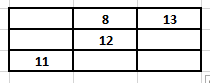(ii)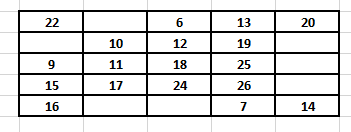Solution:

(i) We know that

Considering diagonal values 13 + 12 + 11 = 36

So we get

No. in the first cell of the first row = 36 – (8 + 13) = 15

No. in the first cell of the second row = 36 – (15 + 11) = 10

No. in the third cell of the second row = 36 – (10 + 12) = 14

No. in the second cell of the third row = 36 – (8 + 12) = 16

No. in the third cell of the third row = 36 – (11 + 16) = 9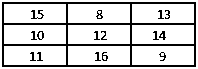(ii) We know that

Considering diagonal values 20 + 19 + 18 + 17 + 16 = 90

So we get

No. in the second cell of the first row = 90 – (22 + 6 + 13 + 20) = 29

No. in the first cell of the second row = 90 – (22 + 9 + 15 + 16) = 28

No. in the fifth cell of the second row = 90 – (28 + 10 + 12 + 19) = 21

No. in the fifth cell of the third row = 90 – (9 + 11 + 18 + 25) = 27

No. in the fifth cell of the fourth row = 90 – (15 + 17 + 24 + 26) = 8

No. in the second cell of the fifth row = 90 – (29 + 10 + 11 + 17) = 23

No. in the third cell of the fifth row = 90 – (6 + 12 + 18 + 24) = 30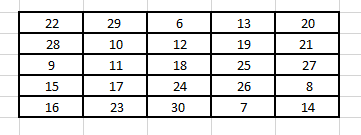2. Perform the following subtractions and check your results by performing corresponding additions:

(i) 57839 – 2983

(ii) 92507 – 10879

(iii) 400000 – 98798

(iv) 5050501 – 969696

(v) 200000 – 97531

(vi) 3030301 – 868686

Solution:

(i) 57839 – 2983

We know that

57839 – 2983 = 54856

54856 + 2983 = 57839

(ii) 92507 – 10879

We know that

92507 – 10879 = 81628

81628 + 10879 = 92507

(iii) 400000 – 98798

We know that

400000 – 98798 = 301202

301202 + 98798 = 400000

(iv) 5050501 – 969696

We know that

5050501 – 969696 = 4080805

4080805 + 969696 = 5050501

(v) 200000 – 97531

We know that

200000 – 97531 = 102469

102469 + 97531 = 200000

(vi) 3030301 – 868686

We know that

3030301 – 868686 = 2161615

2161615 + 868686 = 3030301

3. Replace each * by the correct digit in each of the following:

(i)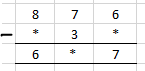(ii)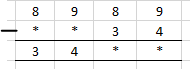(iii)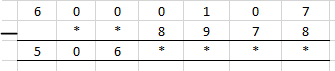(iv)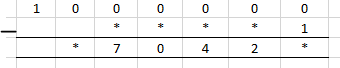(v)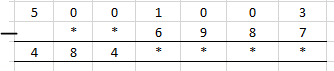(vi)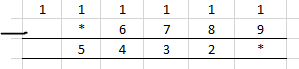Solution:

(i) We know that in the units digit

6 – * = 7 where the value of * is 9 as 1 gets carried from 7 at tens place to 6 at units place

6 at the units place becomes 16 so 16 – 9 = 7

When 7 is reduced by 1 it gives 6 so 6 – 3 = 3

We know that

8 – * = 6 so we get * value as 2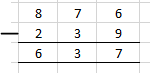(ii) We know that in the units digit

9 – 4 = 5

Tens digit 8 – 3 = 5

So the missing blank can be found by subtracting 3455 from 8989

Difference between them = 3455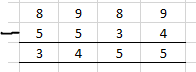(iii) We know that in units digit

17 – 8 = 9

Tens digit = 9 – 7 = 2

So we get

Hundreds place 10 – 9 = 1

Thousands place 9 – 8 = 1

So the addend difference = 5061129

Subtract 5061129 from 6000107 to get addend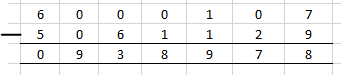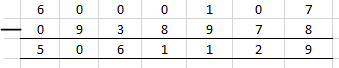(iv) We know that in units digit

10 – 1 = 9

Lakhs place 9 – 0 = 9

So the addend difference = 970429

Subtract 970429 from 1000000 to get the addend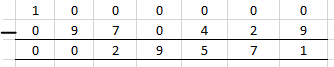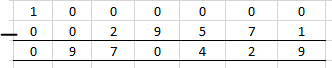(v) We know that in units digit

13 – 7 = 6

Tens digit 9 – 8 = 1

Hundreds place 9 – 9 = 0

Thousands place 10 – 6 = 4

So the addend difference = 4844016

Subtract 4844016 from 5001003 to get the addend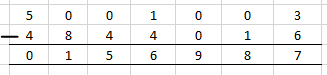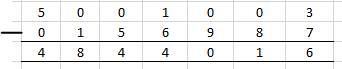(vi) We know that units digit

11 – 9 = 2

So the addend difference = 54322

Subtract 54322 from 111111 to get the addend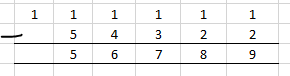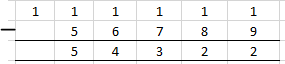4. What is the difference between the largest number of five digits and the smallest number of six digits?

Solution:

99999 is the largest number of five digits

100000 is the largest number of six digits

Difference = 100000 – 99999 = 1

Therefore, 1 is the difference between the largest number of five digits and smallest number of six digits.

5. Find the difference between the largest number of 4 digits and the smallest number of 7 digits.

Solution:

9999 is the largest number of 4 digits

1000000 is the smallest number of 6 digits

Difference = 1000000 – 9999 = 990001

Therefore, 990001 is the difference between the largest number of 4 digits and the smallest number of 7 digits.

6. Rohit deposited Rs 125000 in his savings bank account. Later he withdrew Rs 35425 from it. How much money was left in his account?

Solution:

Money deposited in savings bank account = Rs 125000

Money withdrawn = Rs 35425

So the money which is left out in his account = 125000 – 35425 = Rs 89575

Hence, Rs 89575 is left in his account.

7. The population of a town is 96209. If the number of men is 29642 and that of women is 29167, determine the number of children.

Solution:

Population of a town = 96209

No. of men = 29642

No. of women = 29167

Total number of men and women = 29642 + 29167 = 58809

So the number of children = Population of a town – Total number of men and women

Number of children = 96209 – 58809 = 37400

Hence, there are 37400 children.

8. The digits of 6 and 9 of the number 36490 are interchanged. Find the difference between the original number and the new number.

Solution:

It is given that

Original Number = 39460

Number after interchanging 6 and 9 = 36490

Difference between them = 39460 – 36490 = 2790

Therefore, the difference between the original number and new number is 2970.

9. The population of a town was 59000. In one year it was increased by 4536 due to new births. However, 9218 persons died or left the town during the year. What was the population at the end of the year?

Solution:

Population of a town = 59000

Population increase = 4536

Population decrease = 9218

So the population at the end of year = 59000 + 4536 – 9218 = 54318

Therefore, the population at the end of the year is 54318.

### Exercise 4.3 page: 4.14

1. Fill in the blanks to make each of the following a true statement:

(i) 785 × 0 = …..

(ii) 4567 × 1 = …..

(iii) 475 × 129 = 129 × …..

(iv) ….. × 8975 = 8975 × 1243

(v) 10 × 100 × …. = 10000

(vi) 27 × 18 = 27 × 9 + 27 × ….. + 27 × 5

(vii) 12 × 45 = 12 × 50 – 12 × …..

(viii) 78 × 89 = 78 × 100 – 78 × ….. + 78 × 5

(ix) 66 × 85 = 66 × 90 – 66 × ….. – 66

(x) 49 × 66 + 49 × 34 = 49 × (….. + …..)

Solution:

(i) 785 × 0 = 0

(ii) 4567 × 1 = 4567 based on multiplicative identity

(iii) 475 × 129 = 129 × 475 based on commutativity

(iv) 1243 × 8975 = 8975 × 1243 based on commutativity

(v) 10 × 100 × 10 = 10000

(vi) 27 × 18 = 27 × 9 + 27 × 4 + 27 × 5

(vii) 12 × 45 = 12 × 50 – 12 × 5

(viii) 78 × 89 = 78 × 100 – 78 × 16 + 78 × 5

(ix) 66 × 85 = 66 × 90 – 66 × 4 – 66

(x) 49 × 66 + 49 × 34 = 49 × (66 + 34)

2. Determine each of the following products by suitable rearrangements:

(i) 2 × 1497 × 50

(ii) 4 × 358 × 25

(iii) 495 × 625 × 16

(iv) 625 × 20 × 8 × 50

Solution:

(i) 2 × 1497 × 50

It can be written as

2 × 1497 × 50 = (2 × 50) × 1497

= 100 × 1497

= 149700

(ii) 4 × 358 × 25

It can be written as

4 × 358 × 25 = (4 × 25) × 358

= 100 × 358

= 35800

(iii) 495 × 625 × 16

It can be written as

495 × 625 × 16 = (625 × 16) × 495

= 10000 × 495

= 4950000

(iv) 625 × 20 × 8 × 50

It can be written as

625 × 20 × 8 × 50 = (625 × 8) × (20 × 50)

= 5000 × 1000

= 5000000

3. Using distributivity of multiplication over addition of whole numbers, find each of the following products:

(i) 736 × 103

(ii) 258 × 1008

(iii) 258 × 1008

Solution:

(i) 736 × 103

It can be written as

= 736 × (100 + 3)

By using distributivity of multiplication over addition of whole numbers

= (736 × 100) + (736 × 3)

On further calculation

= 73600 + 2208

We get

= 75808

(ii) 258 × 1008

It can be written as

= 258 × (1000 + 8)

By using distributivity of multiplication over addition of whole numbers

= (258 × 1000) + (258 × 8)

On further calculation

= 258000 + 2064

We get

= 260064

(iii) 258 × 1008

It can be written as

= 258 × (1000 + 8)

By using distributivity of multiplication over addition of whole numbers

= (258 × 1000) + (258 × 8)

On further calculation

= 258000 + 2064

We get

= 260064

4. Find each of the following products:

(i) 736 × 93

(ii) 816 × 745

(iii) 2032 × 613

Solution:

(i) 736 × 93

It can be written as

= 736 × (100 – 7)

By using distributivity of multiplication over subtraction of whole numbers

= (736 × 100) – (736 × 7)

On further calculation

= 73600 – 5152

We get

= 68448

(ii) 816 × 745

It can be written as

= 816 × (750 – 5)

By using distributivity of multiplication over subtraction of whole numbers

= (816 × 750) – (816 × 5)

On further calculation

= 612000 – 4080

We get

= 607920

(iii) 2032 × 613

It can be written as

= 2032 × (600 + 13)

By using distributivity of multiplication over addition of whole numbers

= (2032 × 600) + (2032 × 13)

On further calculation

= 1219200 + 26416

We get

= 1245616

5. Find the values of each of the following using properties:

(i) 493 × 8 + 493 × 2

(ii) 24579 × 93 + 7 × 24579

(iii) 1568 × 184 – 1568 × 84

(iv) 15625 × 15625 – 15625 × 5625

Solution:

(i) 493 × 8 + 493 × 2

It can be written as

= 493 × (8 + 2)

By using distributivity of multiplication over addition of whole numbers

= 493 × 10

On further calculation

= 4930

(ii) 24579 × 93 + 7 × 24579

It can be written as

= 24579 × (93 + 7)

By using distributivity of multiplication over addition of whole numbers

= 24579 × 100

On further calculation

= 2457900

(iii) 1568 × 184 – 1568 × 84

It can be written as

= 1568 × (184 – 84)

By using distributivity of multiplication over subtraction of whole numbers

= 1568 × 100

On further calculation

= 156800

(iv) 15625 × 15625 – 15625 × 5625

It can be written as

= 15625 × (15625 – 5625)

By using distributivity of multiplication over subrtaction of whole numbers

= 15625 × 10000

On further calculation

= 156250000

6. Determine the product of:

(i) the greatest number of four digits and the smallest number of three digits.

(ii) the greatest number of five digits and the greatest number of three digits.

Solution:

(i) We know that

Largest four digit number = 9999

Smallest three digit number = 100

Product of both = 9999 × 100 = 999900

Hence, the product of the greatest number of four digits and the smallest number of three digits is 999900.

(ii) We know that

Largest five digit number = 99999

Largest three digit number = 999

Product of both = 99999 × 999

It can be written as

= 99999 × (1000 – 1)

By using distributivity of multiplication over addition subrtaction of whole numbers

= (99999 × 1000) – (99999 × 1)

On further calculation

= 99999000 – 99999

We get

= 99899001

7. In each of the following, fill in the blanks, so that the statement is true:

(i) (500 + 7) (300 – 1) = 299 × …..

(ii) 888 + 777 + 555 = 111 × …..

(iii) 75 × 425 = (70 + 5) (….. + 85)

(iv) 89 × (100 – 2) = 98 × (100 – …..)

(v) (15 + 5) (15 – 5) = 225 – …..

(vi) 9 × (10000 + …..) = 98766

Solution:

(i) By considering LHS

(500 + 7) (300 – 1)

We get

= 507 × 299

By using commutativity

= 299 × 507

(ii) By considering LHS

888 + 777 + 555

We get

= 111 (8 + 7 + 5)

By using distributivity

= 111 × 20

(iii) By considering LHS

75 × 425

We get

= (70 + 5) × 425

It can be written as

= (70 + 5) (340 + 85)

(iv) By considering LHS

89 × (100 – 2)

We get

= 89 × 98

It can be written as

= 98 × 89

By using commutativity

= 98 × (100 – 11)

(v) By considering LHS

(15 + 5) (15 – 5)

We get

= 20 × 10

On further calculation

= 200

It can be written as

= 225 – 25

(vi) By considering LHS

9 × (10000 + 974) = 98766

8. A dealer purchased 125 colour television sets. If the cost of each set is Rs 19820, determine the cost of all sets together.

Solution:

It is given that

Cost of each television set = Rs 19820

So we get

Cost of 125 television sets = 19820 × 125

It can be written as

= 19820 × (100 + 25)

By using distributivity of multiplication over addition of whole numbers

= (19820 × 100) + (19820 × 25)

On further calculation

= 1982000 + 495500

So we get

= Rs 2477500

9. The annual fee charged from a student of class VI in a school is Rs 8880. If there are, in all, 235 students in class VI, find the total collection.

Solution:

Annual fee per student = Rs 8880

So we get

Annual fee charged for 235 students = 8880 × 235 = 2086800

Therefore, the total collection is Rs 2086800.

10. A group housing society constructed 350 flats. If the cost of construction for each flat is Rs 993570, what is the total cost of construction of all the flats.

Solution:

Cost of construction for each flat = Rs 993570

Number of flats constructed = 350

So we get

Cost of construction of 350 flats = 993570 × 350 = Rs 347749500

Therefore, the total cost of construction of all the flats is Rs 347749500.

11. The product of two whole numbers is zero. What do you conclude?

Solution:

The product of two whole numbers is zero, which means that at least one number or both of them are zero.

12. What are the whole numbers which when multiplied with itself gives the same number?

Solution:

Two numbers when multiplied with itself gives the same number.

For example: 0 × 0 = 0 and 1 × 1 = 1

13. In a large housing complex, there are 15 small buildings and 22 large building. Each of the large buildings has 10 floors with 2 apartments on each floor. Each of the small buildings has 12 floors with 3 apartments on each floor. How many apartments are there in all.

Solution:

It is given that

No. of large buildings = 22

No. of small buildings = 15

No. of floors in 1 large building = 10

No. of apartments on 1 floor = 2

So total apartment in 1 large building = 10 × 2 = 20

The same way

No. of apartments in 1 small building = 12 × 3 = 36

So the total apartment in entire housing complex = (22 × 20) + (15 × 36) = 440 + 540 = 980

Therefore, there are 980 apartments in all.

### Exercise 4.4 page: 4.19

1. Does there exist a whole number a such that a ÷ a = a?

Solution:

Yes. There exists a whole number ‘a’ such that a ÷ a = a.

We know that the whole number is 1 where 1 ÷ 1 = 1.

2. Find the value of:

(i) 23457 ÷ 1

(ii) 0 ÷ 97

(iii) 476 + (840 ÷ 84)

(iv) 964 – (425 ÷ 425)

(v) (2758 ÷ 2758) – (2758 ÷ 2758)

(vi) 72450 ÷ (583 – 58)

Solution:

(i) 23457 ÷ 1

By division

23457 ÷ 1 = 23457

(ii) 0 ÷ 97

By division

0 ÷ 97 = 0

(iii) 476 + (840 ÷ 84)

On further calculation

476 + (840 ÷ 84) = 476 + 10

= 486

(iv) 964 – (425 ÷ 425)

On further calculation

964 – (425 ÷ 425) = 964 – 1

= 963

(v) (2758 ÷ 2758) – (2758 ÷ 2758)

On further calculation

(2758 ÷ 2758) – (2758 ÷ 2758) = 1 – 1

= 0

(vi) 72450 ÷ (583 – 58)

On further calculation

72450 ÷ (583 – 58) = 72450 ÷ 525

= 138

3. Which of the following statements are true:

(i) 10 ÷ (5 × 2) = (10 ÷ 5) × (10 ÷ 2)

(ii) (35 – 14) ÷ 7 = 35 ÷ 7 – 14 ÷ 7

(iii) 35 – 14 ÷ 7 = 35 ÷ 7 – 14 ÷ 7

(iv) (20 – 5) ÷ 5 = 20 ÷ 5 – 5

(v) 12 × (14 ÷ 7) = (12 × 14) ÷ (12 × 7)

(vi) (20 ÷ 5) ÷ 2 = (20 ÷ 2) ÷ 5

Solution:

(i) False.

We know that

LHS = 10 ÷ (5 × 2)

So we get

= 10 ÷ 10

= 1

RHS = (10 ÷ 5) × (10 ÷ 2)

So we get

= 2 × 5

= 10

(ii) True.

We know that

LHS = (35 – 14) ÷ 7

So we get

= 21 ÷ 7

= 3

RHS = 35 ÷ 7 – 14 ÷ 7

So we get

= 5 – 2

= 3

(iii) False.

We know that

LHS = 35 – 14 ÷ 7

So we get

= 35 – 2

= 33

RHS = 35 ÷ 7 – 14 ÷ 7

So we get

= 5 – 2

= 3

(iv) False.

We know that

LHS = (20 – 5) ÷ 5

So we get

= 15 ÷ 5

= 3

RHS = 20 ÷ 5 – 5

So we get

= 4 – 5

= – 1

(v) False.

We know that

LHS = 12 × (14 ÷ 7)

So we get

= 12 × 2

= 24

RHS = (12 × 14) ÷ (12 × 7)

So we get

= 168 ÷ 84

= 2

(vi) True.

We know that

LHS = (20 ÷ 5) ÷ 2

So we get

= 4 ÷ 2

= 2

RHS = (20 ÷ 2) ÷ 5

So we get

= 10 ÷ 5

= 2

4. Divide and check the quotient and remainder:

(i) 7772 ÷ 58

(ii) 6906 ÷ 35

(iii) 16135 ÷ 875

(iv) 16025 ÷ 1000

Solution:

(i) 7772 ÷ 58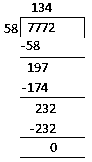So we get 7772 ÷ 58 = 134

By verifying

We know that

Dividend = Divisor × Quotient + Remainder

By substituting values

7772 = 58 × 134 + 0

So we get

7772 = 7772

LHS = RHS

(ii) 6906 ÷ 35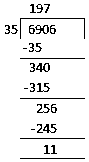So we get quotient = 197 and remainder = 11

By verifying

We know that

Dividend = Divisor × Quotient + Remainder

By substituting values

6906 = 35 × 197 + 11

On further calculation

6906 = 6895 + 11

We get

6906 = 6906

LHS = RHS

(iii) 16135 ÷ 875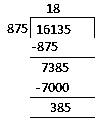So we get quotient = 18 and remainder = 385

By verifying

We know that

Dividend = Divisor × Quotient + Remainder

By substituting values

16135 = 875 × 18 + 385

On further calculation

16135 = 15750 + 385

We get

16135 = 16135

LHS = RHS

(iv) 16025 ÷ 1000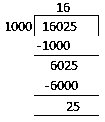So we get quotient = 16 and remainder = 25

By verifying

We know that

Dividend = Divisor × Quotient + Remainder

By substituting values

16025 = 1000 × 16 + 25

On further calculation

16025 = 16000 + 25

We get

16025 = 16025

LHS = RHS

5. Find a number which when divided by 35 gives the quotient 20 and remainder 18.

Solution:

We know that

Dividend = Divisor × Quotient + Remainder

By substituting values

Dividend = 35 × 20 + 18

On further calculation

Dividend = 700 + 18

So we get

Dividend = 718

6. Find the number which when divided by 58 gives a quotient 40 and remainder 31.

Solution:

We know that

Dividend = Divisor × Quotient + Remainder

By substituting values

Dividend = 58 × 40 + 31

On further calculation

Dividend = 2320 + 31

So we get

Dividend = 2351

7. The product of two numbers is 504347. If one of the numbers is 1591, find the other.

Solution:

The product of two numbers = 504347

One of the numbers = 1591

Consider A as the number

A × 1591 = 504347

So by division

A = 317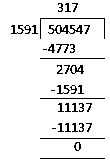8. On dividing 59761 by a certain number, the quotient is 189 and the remainder is 37. Find the divisor.

Solution:

It is given that

Dividend = 59761

Quotient = 189

Remainder = 37

Consider Divisor = A

We know that

Dividend = Divisor × Quotient + Remainder

By substituting values

59761 = A × 189 + 37

On further calculation

59761 – 37 = A × 189

So we get

59724 = A × 189

By division

A = 316

9. On dividing 55390 by 299, the remainder is 75. Find the quotient.

Solution:

It is given that

Dividend = 55390

Divisor = 299

Remainder = 75

Consider Quotient = A

We know that

Dividend = Divisor × Quotient + Remainder

By substituting values

55390 = 299 × A + 75

On further calculation

55390 – 75 = A × 299

So we get

55315 = A × 299

By division

A = 185

### Exercise 4.5 page: 4.23

1. Without drawing a diagram, find

(i) 10th square number

(ii) 6th triangular number

Solution:

(i) 10th square number

The square number can be remembered using the following rule

Nth square number = n × n

So the 10th square number = 10 × 10 = 100

(ii) 6th triangular number

The triangular number can be remembered using the following rule

Nth triangular number = n × (n + 1)/ 2

So the 6th triangular number = 6 × (6 + 1)/ 2 = 21

2. (i) Can a rectangular number also be a square number?

(ii) Can a triangular number also be a square number?

Solution:

(i) Yes. A rectangular number can also be a square number.

Example – 16 is a rectangular number which can also be a square number.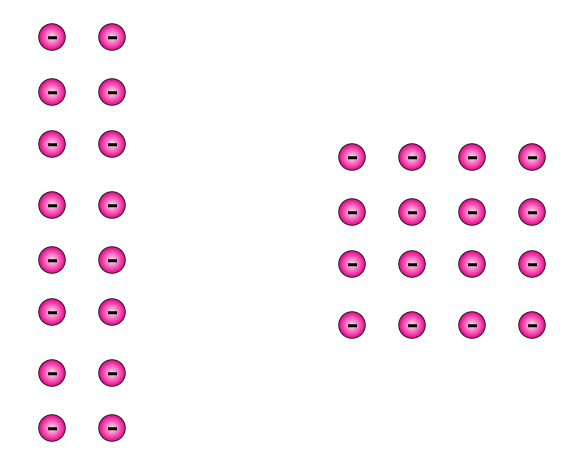(ii) Yes. A triangular number can also be a square number.

Example – 1 is a triangular number which can also be a square number.

3. Write the first four products of two numbers with difference 4 starting from in the following order:

1, 2, 3, 4, 5, 6, ………

Identify the pattern in the products and write the next three products.

Solution:

We know that

1 × 5 = 5

2 × 6 = 12

3 × 7 = 21

4 × 8 = 32

So the first four products of two numbers with difference 4

5 – 1 = 4

6 – 2 = 4

7 – 3 = 4

8 – 4 = 4

4. Observe the pattern in the following and fill in the blanks:

9 × 9 + 7 = 88

98 × 9 + 6 = 888

987 × 9 + 5 = 8888

9876 × 9 + 4 = ………

98765 × 9 + 3 = ………

987654 × 9 + 2 = ……….

9876543 × 9 + 1 = ……….

Solution:

9 × 9 + 7 = 88

98 × 9 + 6 = 888

987 × 9 + 5 = 8888

9876 × 9 + 4 = 88888

98765 × 9 + 3 = 888888

987654 × 9 + 2 = 8888888

9876543 × 9 + 1 = 88888888

5. Observe the following pattern and extend it to three more steps:

6 × 2 – 5 = 7

7 × 3 – 12 = 9

8 × 4 – 21 = 11

9 × 5 – 32 = 13

….. × ……. – ….. = …….

….. × ……. – ….. = …….

….. × ……. – ….. = …….

Solution:

6 × 2 – 5 = 7

7 × 3 – 12 = 9

8 × 4 – 21 = 11

9 × 5 – 32 = 13

10 × 6 – 45 = 15

11 × 7 – 60 = 17

12 × 8 – 77 = 19

6. Study the following pattern:

1 + 3 = 2 × 2

1 + 3 + 5 = 3 × 3

1 + 3 + 5 + 7 = 4 × 4

1 + 3 + 5 + 7 + 9 = 5 × 5

By observing the above pattern, find

(i) 1 + 3 + 5 + 7 + 9 + 11

(ii) 1 + 3 + 5 + 7 + 9 + 11 + 13 + 15

(iii) 21 + 23 + 25 + ….. + 51

Solution:

(i) 1 + 3 + 5 + 7 + 9 + 11

By using the pattern

1 + 3 + 5 + 7 + 9 + 11 = 6 × 6

= 36

(ii) 1 + 3 + 5 + 7 + 9 + 11 + 13 + 15

By using the pattern

1 + 3 + 5 + 7 + 9 + 11 + 13 + 15 = 8 × 8

= 64

(iii) 21 + 23 + 25 + ….. + 51

We know that

21 + 23 + 25 + ….. + 51 can be written as (1 + 3 + 5 + 7 + ….. + 49 + 51) – (1 + 3 + 5 + …… + 17 + 19)

By using the pattern

(1 + 3 + 5 + 7 + ….. + 49 + 51) = 26 × 26 = 676

(1 + 3 + 5 + …… + 17 + 19) = 10 × 10 = 100

So we get

21 + 23 + 25 + ….. + 51 = 676 – 100 = 576

7. Study the following pattern:

1 × 1 + 2 × 2 = (2 × 3 × 5)/ 6

1 × 1 + 2 × 2 + 3 × 3 = (3 × 4 × 7) / 6

1 × 1 + 2 × 2 + 3 × 3 + 4 × 4 = (4 × 5 × 9)/ 6

By observing the above pattern, write next two steps.

Solution:

By using the pattern

1 × 1 + 2 × 2 + 3 × 3 + 4 × 4 + 5 × 5

On further calculation

= (5 × 6 × 11)/6

So we get

= 55

By using the pattern

1 × 1 + 2 × 2 + 3 × 3 + 4 × 4 + 5 × 5 + 6 × 6

On further calculation

= (6 × 7 × 13)/6

So we get

= 91

8. Study the following pattern:

1 = (1 × 2)/ 2

1 + 2 = (2 × 3)/ 2

1 + 2 + 3 = (3 × 4)/ 2

1 + 2 + 3 + 4 = (4 × 5)/ 2

By observing the above pattern, find

(i) 1 + 2 + 3 + 4 + 5 + 6 + 7 + 8 + 9 + 10

(ii) 50 + 51 + 52 + ……. + 100

(iii) 2 + 4 + 6 + 8 + 10 + …….. + 100

Solution:

(i) 1 + 2 + 3 + 4 + 5 + 6 + 7 + 8 + 9 + 10

We get

= (10 × 11)/2

On further calculation

= 55

(ii) 50 + 51 + 52 + ……. + 100

We can write it as

(1 + 2 + 3 + …… + 99 + 100) – (1 + 2 + 3 + 4 + ….. + 47 + 49)

So we get

(1 + 2 + 3 + …… + 99 + 100) = (100 × 101)/2

(1 + 2 + 3 + 4 + ….. + 47 + 49) = (49 × 50)/2

By substituting the values

50 + 51 + 52 + ……. + 100 = (100 × 101)/2 + (49 × 50)/2

On further calculation

= 5050 – 1225

We get

= 3825

(iii) 2 + 4 + 6 + 8 + 10 + …….. + 100

We can write it as

2 (1 + 2 + 3 + 4 + …… + 49 + 50)

So we get

= 2 × (50 × 51)/2

On further calculation

= 2 × (1275)

We get

= 2550

### Objective Type Questions PAGE: 4.24

Mark the correct alternative in each of the following:

1. Which one of the following is the smallest whole number?
(a) 1                     (b) 2                         (c) 0                                     (d) None of these

Solution:

The option (c) is correct answer.

We know that the set of whole numbers is {0, 1, 2, 3, 4 …}.

Hence, the smallest whole number is 0.

2. Which one of the following is the smallest even whole number?
(a) 0                               (b) 1                                   (c) 2                                      (d) None of these

Solution:

The option (c) is correct answer.

We know that the natural numbers along with 0 form the collection of whole numbers.

Hence, the numbers 0, 1, 2, 3, 4 … form the collection of whole numbers.

So the number which is divisible by 2 is an even number and 2 is the smallest even number.

3. Which one of the following is the smallest odd whole number?
(a) 0                               (b) 1                                   (c) 3                                      (d) 5

Solution:

The option (b) is correct answer.

We know that the natural numbers along with 0 form the collection of whole numbers.

Hence, the numbers 0, 1, 2, 3, 4 … form the collection of whole numbers.

So the natural number which is not divisible by 2 is called an odd whole number and 1 is the smallest odd whole number.

4. How many whole numbers are between 437 and 487?
(a) 50                               (b) 49                                   (c) 51                                      (d) None of these

Solution:

The option (b) is correct answer.

We know that the whole numbers between 437 and 487 are 438, 439, 440, 441, …, 484, 485 and 486.

In order to find the required number of whole numbers subtract 437 from 487 and then subtract again 1.

Hence, there are (487 − 437) − 1 whole numbers lying between 437 and 487.

So we get (487 − 437) − 1 = 50 − 1 = 49

5. The product of the successor of 999 and the predecessor of 1001 is
(a) one lakh                            (b) one billion                               (c) one million                                (d) one crore

Solution:

The option (c) is correct answer.

We know that the successor of 999 = 999 + 1 = 1000

So the predecessor of 1001 = 1001 − 1 = 1000

It can be written as

Product of them = (Successor of 999) × (Predecessor of 1001)

By substituting the values

Product of them = 1000 × 1000 = 1000000 = one million

6. Which one of the following whole numbers does not have a predecessor?
(a) 1                            (b) 0                              (c) 2                               (d) None of these

Solution:

The option (b) is correct answer.

We know that the numbers 0, 1, 2, 3, 4 … form the collection of whole numbers.

Hence, the smallest whole number is 0 which does not have a predecessor.

7. The number of whole numbers between the smallest whole number and the greatest 2-digit number is
(a) 101                            (b) 100                              (c) 99                               (d) 98

Solution:

The option (d) is correct answer.

We know that the smallest whole number = 0

So the greatest 2 digit whole number = 99

Whole numbers which lie between 0 and 99 are 1, 2, 3, 4,…, 97, 98.

In order to find the number of whole numbers between 0 and 99, first subtract 1 from the difference of 0 and 99.

So the number of whole numbers between 0 and 99 = (99 − 0) − 1 = 99 − 1 = 98

8. If n is a whole number such that n + n = n, then n =?
(a) 1                            (b) 2                              (c) 3                               (d) None of these

Solution:

The option (d) is correct answer.

We know that 0 + 0 = 0, 1 + 1 = 2, 2 + 2 = 4….

Hence, the statement n + n = n is true only when n = 0.

9. The predecessor of the smallest 3-digit number is
(a) 999                            (b) 99                              (c) 100                               (d) 101

Solution:

The option (b) is correct answer.

We know that the smallest 3 digit number = 100

So the predecessor of 3 digit number = 100 − 1 = 99

10. The least number of 4-digits which is exactly divisible by 9 is
(a) 1008                            (b) 1009                              (c) 1026                               (d) 1018

Solution:

The option (a) is correct answer.

We know that the least 4-digit number = 1000

Hence, the least 4-digits which is exactly divisible by 9 is 1000 + (9 − 1) = 1008

11. The number which when divided by 53 gives 8 as quotient and 5 as remainder is
(a) 424                 (b) 419                       (c) 429                              (d) None of these

Solution:

The option (c) is correct answer.

It is given that

Divisor = 53, Quotient = 8 and Remainder = 5.

By using the relation we get

Dividend = Divisor × Quotient + Remainder

By substituting the values

Dividend = 53 × 8 + 5 = 424 + 5 =429

Hence, the required number is 429.

12. The whole number n satisfying n + 35 = 101 is
(a) 65                 (b) 67                       (c) 64                              (d) 66

Solution:

The option (d) is correct answer.

It is given that

n + 35 = 101

By adding − 35 on both sides

n + 35 + (− 35) = 101 + (− 35)

On further calculation

n + 0 = 66

So we get

n = 66

13. The 4 × 378 × 25 is
(a) 37800                 (b) 3780                       (c) 9450                              (d) 30078

Solution:

The option (a) is correct answer.

We can write it as

4 × 378 × 25 = 4 × 25 × 378

On further calculation

4 × 378 × 25 = 100 × 378 = 37800

14. The value of 1735 × 1232 − 1735 × 232 is
(a) 17350                 (b) 173500                       (c) 1735000                              (d) 173505

Solution:

The option (c) is correct answer.

By using the distributive law of multiplication over subtraction

1735 × 1232 − 1735 × 232 = 1735(1232 − 232)

On further calculation

1735 × 1232 − 1735 × 232 = 1735 × 1000 = 1735000

15. The value of 47 × 99 is
(a) 4635                 (b) 4653                       (c) 4563                              (d) 6453

Solution:

The option (b) is correct answer.

It can be written as

99 = 100 − 1

So we get

47 × 99 = 47 × (100 − 1)

On further calculation

47 × 99 = 47× 100 – 47 = 4700 – 47 = 4653

Hence, the value of 47 × 99 is 4653.

### RD Sharma Solutions for Class 6 Maths Chapter 4: Operations on Whole Numbers

Chapter 4, Operations on Whole Numbers, has 5 exercises based on addition, subtraction, division and multiplication operations on whole numbers. Some of the important topics in RD Sharma Solutions Chapter 4 are given below:

• Introduction